# Theoretical background for IDEA StatiCa Detail

Tento článek je dostupný také v dalších jazycích:
$$The theoretical background is based on COMPATIBLE STRESS FIELD DESIGN OF STRUCTURAL CONCRETE (Kaufmann et al., 2020) 1 General introduction The design and assessment of concrete elements are normally performed at the sectional (1D-element) or point (2D-element) level. This procedure is described in all standards for structural design, e.g., in (EN 1992-1-1), and it is used in everyday structural engineering practice. However, it is not always known or respected that the procedure is only acceptable in areas where Bernoulli-Navier hypothesis of plane strain distribution applies (referred to as B-regions). The places where this hypothesis does not apply are called discontinuity or disturbed regions (D-Regions). Examples of B and D regions of 1D-elements are given in (Fig. 1). These are, e.g., bearing areas, parts where concentrated loads are applied, locations where an abrupt change in the cross-section occurs, openings, etc. When designing concrete structures, we meet a lot of other D-Regions such as walls, bridge diaphragms, corbels, etc.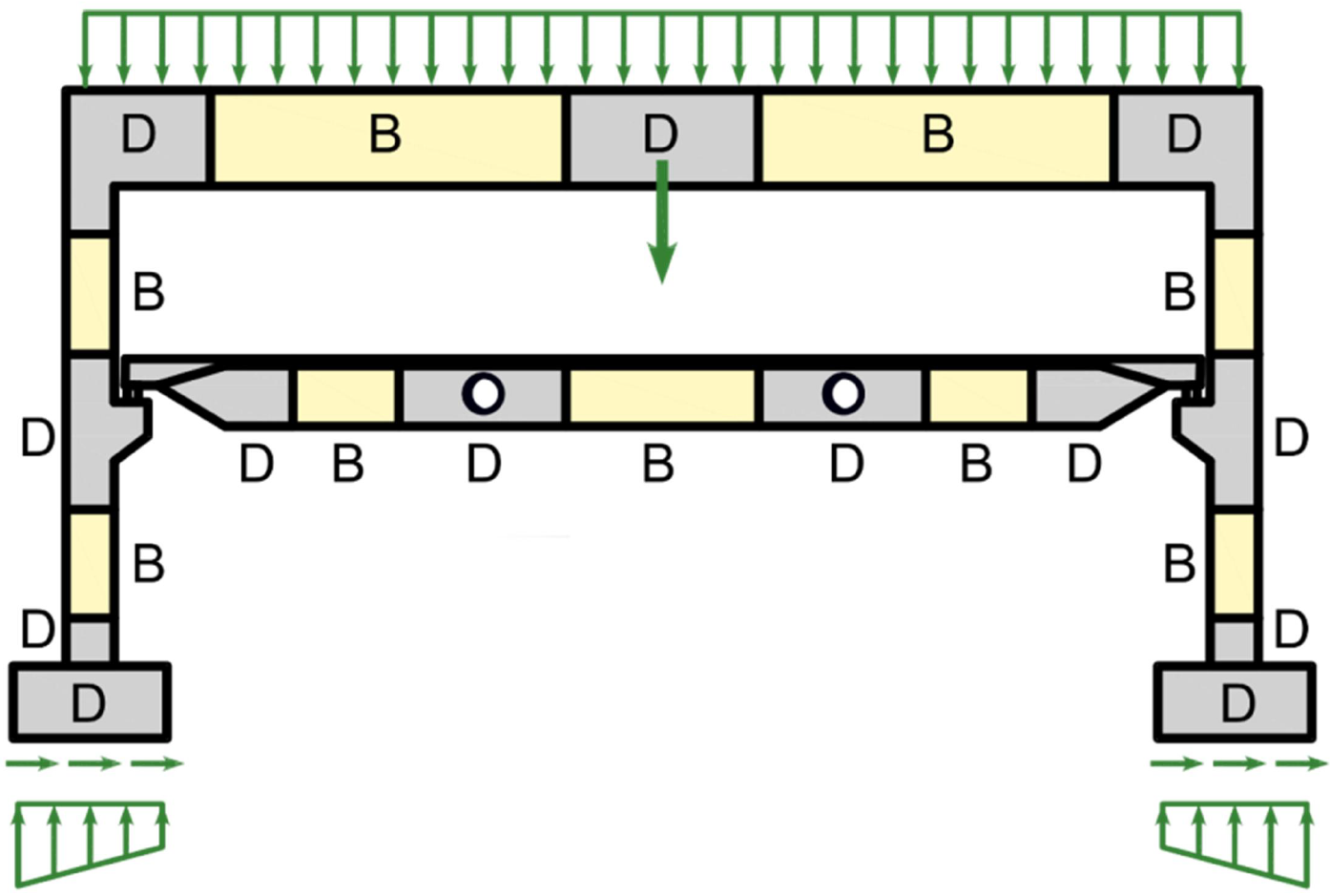$\textsf{\textit{\footnotesize{Fig. 1\qquad Discontinuity regions (Navrátil et al. 2017)}}}$In the past, semi-empirical design rules were used for dimensioning discontinuity regions. Fortunately, these rules have been largely superseded over the past decades by strut-and-tie models (Schlaich et al., 1987) and stress fields (Marti 1985), which are featured in current design codes and frequently used by designers today. These models are mechanically consistent and powerful tools. Note that stress fields can generally be continuous or discontinuous and that strut-and-tie models are a special case of discontinuous stress fields.Despite the evolution of computational tools over the past decades, Strut-and-Tie models are essentially still used as hand calculations. Their application for real-world structures is tedious and time-consuming since iterations are required, and several load cases need to be considered. Furthermore, this method is not suitable for verifying serviceability criteria (deformations, crack widths, etc.).The interest of structural engineers in a reliable and fast tool to design D-regions led to the decision to develop the new Compatible Stress Field Method, a method for computer-aided stress field design that allows the automatic design and assessment of structural concrete members subjected to in-plane loading.The Compatible Stress Field Method is a continuous FE-based stress field analysis method in which classic stress field solutions are complemented with kinematic considerations, i.e., the state of strain is evaluated throughout the structure. Hence, the effective compressive strength of concrete can be automatically computed based on the state of transverse strain in a similar manner as in compression field analyses that account for compression softening (Vecchio and Collins 1986; Kaufmann and Marti 1998) and the EPSF method (Fernández Ruiz and Muttoni 2007). Moreover, the CSFM considers tension stiffening, providing realistic stiffnesses to the elements, and covers all design code prescriptions (including serviceability and deformation capacity aspects) not consistently addressed by previous approaches. The CSFM uses common uniaxial constitutive laws provided by design standards for concrete and reinforcement. These are known at the design stage, which allows the partial safety factor method to be used. Hence, designers do not have to provide additional, often arbitrary material properties as are typically required for non-linear FE-analyses, making the method perfectly suitable for engineering practice.To foster the use of computer-aided stress fields by structural engineers, these methods should be implemented in user-friendly software environments. To this end, the CSFM has been implemented in IDEA StatiCa Detail; a new user-friendly commercial software developed jointly by ETH Zurich and the software company IDEA StatiCa in the framework of the DR-Design Eurostars-10571 project.1.1 Main assumptions and limitationsThe CSFM assumes fictitious, rotating, stress-free cracks that open without slip (Fig. 2a), and considers the equilibrium at the cracks together with the average strains of the reinforcement. Hence, the model considers maximum concrete (σc3r) and reinforcement stresses (σsr) at the cracks while neglecting the concrete tensile strength (σc1r = 0), except for its stiffening effect on the reinforcement. The consideration of tension stiffening allows the average reinforcement strains (εm) to be simulated.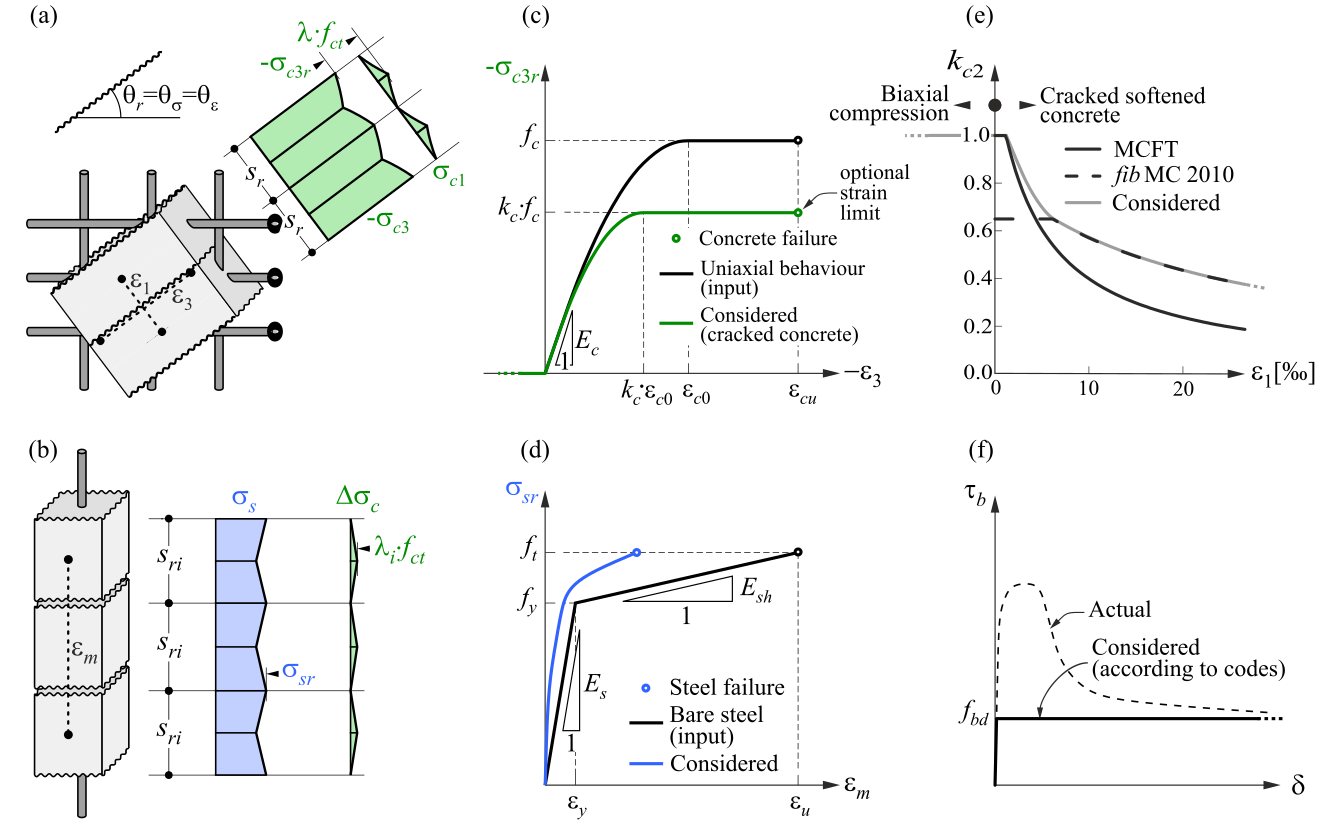$$\textsf{\textit{\footnotesize{Fig. 2\qquad Basic assumptions of the CSFM: (a) principal stresses in concrete; (b) stresses in the reinforcement direction;}}}$$ $$\textsf{\textit{\footnotesize{(c) stress-strain diagram of concrete in terms of maximum stresses with consideration of compression softening;}}}$$ $$\textsf{\textit{\footnotesize{(d) stress-strain diagram of reinforcement in terms of stresses at cracks and average strains; (e) compression softening}}}$$ $$\textsf{\textit{\footnotesize{law; (f) bond shear stress-slip relationship for anchorage length verifications.}}}$$Despite their simplicity, similar assumptions have been demonstrated to yield accurate predictions for reinforced members subjected to in-plane loading (Kaufmann 1998; Kaufmann and Marti 1998) if the provided reinforcement avoids brittle failures at cracking. Furthermore, the non-consideration of any contribution of the tensile strength of concrete to the ultimate load is consistent with the principles of modern design codes, which are mostly based on plasticity theory.However, the CSFM is not suited for slender elements without transverse reinforcement, since relevant mechanisms for such elements as aggregate interlock, residual tensile stresses at the crack tip and dowel action – all of them relying directly or indirectly on the tensile strength of the concrete – are disregarded. While some design standards allow the design of such elements based on semi-empirical provisions, the CSFM is not intended for this type of potentially brittle structure.1.2 Constitutive model1.2.1 ConcreteThe concrete model implemented in the CSFM is based on the uniaxial compression constitutive laws prescribed by design codes for the design of cross-sections, which only depend on compressive strength. The parabola-rectangle diagram specified in EN 1992-1-1 (Fig. 2c) is used by default in the CSFM, but designers can also choose a more simplified elastic ideal plastic relationship. When assessing according to ACI 3018-04, it is possible to use only the parabola-rectangle stress-strain diagram. As previously mentioned, the tensile strength is neglected, as it is in classic reinforced concrete design.The effective compressive strength is automatically evaluated for cracked concrete based on the principal tensile strain (ε1) by means of the kc2 reduction factor, as shown in Fig. 2c and e. The implemented reduction relationship (Fig. 2e) is a generalization of the fib Model Code 2010 proposal for shear verifications, which contains a limiting value of 0.65 for the maximum ratio of effective concrete strength to concrete compressive strength, which is not applicable to other loading cases.Using default settings, the current implementation of the CSFM in IDEA StatiCa Detail does not consider an explicit failure criterion in terms of strains for concrete in compression (i.e., it considers an infinitely plastic branch after the peak stress is reached). This simplification does not allow the deformation capacity of structures failing in compression to be verified. However, their ultimate capacity is properly predicted when, in addition to the factor of cracked concrete (kc2) defined in (Fig. 2e), the increase in the brittleness of concrete as its strength rises is considered by means of the $$\eta_{fc}$$ reduction factor defined in fib Model Code 2010 as follows:$f_{ck,red} = k_c \cdot f_{ck} = \eta _{fc} \cdot k_{c2} \cdot f_{ck}$${\eta _{fc}} = {\left( {\frac{{30}}{{{f_{ck}}}}} \right)^{\frac{1}{3}}} \le 1$where:kc is the global reduction factor of the compressive strengthkc2 is the reduction factor due to the presence of transverse crackingfck is the concrete cylinder characteristic strength (in MPa for the definition of $$\eta_{fc}$$).1.2.2 ReinforcementBy default, the idealized bilinear stress-strain diagram for the bare reinforcing bars typically defined by design codes (Fig. 2d) is considered. The definition of this diagram only requires the basic properties of the reinforcement to be known during the design phase (strength and ductility class). A user-defined stress-strain relationship can also be defined.Tension stiffening is accounted for by modifying the input stress-strain relationship of the bare reinforcing bar in order to capture the average stiffness of the bars embedded in the concrete (εm) (Section 1.2.4).1.2.3 Verification of anchorage lengthBond-slip between reinforcement and concrete is introduced in the finite element model for ultimate limit state load cases by considering the simplified rigid-perfectly plastic constitutive relationship presented in Fig. 2f, with fbd being the design value of the ultimate bond stress specified by the design code for the specific bond conditions.This is a simplified model with the sole purpose of verifying bond prescriptions according to design codes (i.e., anchorage of reinforcement). The reduction of the anchorage length when using hooks, loops, and similar bar shapes can be considered by defining a certain capacity at the end of the reinforcement, as will be described in Section 3.5.3. It should be noted that a different bond shear stress-slip model is considered for tension stiffening and crack width calculations. In such cases, the model considers average bond shear stresses instead of characteristic values to capture the average behavior of the elements.1.2.4 Tension stiffeningThe implementation of tension stiffening distinguishes between cases of stabilized and non-stabilized crack patterns. In both cases, the concrete is considered fully cracked before loading by default.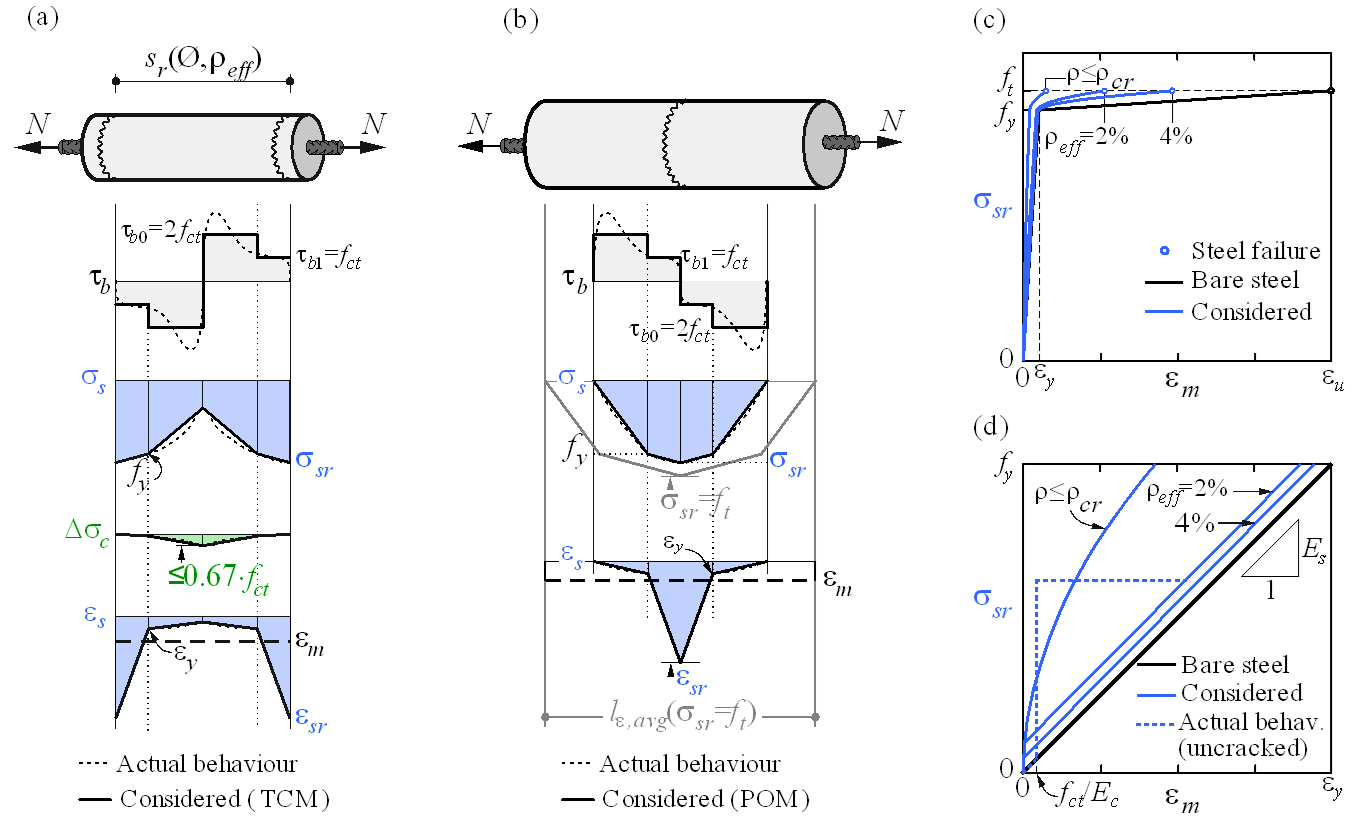$$\textsf{\textit{\footnotesize{Fig. 3\qquad Tension stiffening model: (a) tension chord element for stabilized cracking with distribution of bond shear,}}}$$ $$\textsf{\textit{\footnotesize{steel and concrete stresses, and steel strains between cracks, considering average crack spacing); (b) pull-out assumption}}}$$ $$\textsf{\textit{\footnotesize{for non-stabilized cracking with distribution of bond shear and steel stresses and strains around the crack; (c) resulting}}}$$ $$\textsf{\textit{\footnotesize{tension chord behavior in terms of reinforcement stresses at the cracks and average strains for European B500B steel;}}}$$ $$\textsf{\textit{\footnotesize{(d) detail of the initial branches of the tension chord response.}}}$$Stabilized crackingIn fully developed crack patterns, tension stiffening is introduced using the Tension Chord Model (TCM) (Marti et al. 1998; Alvarez 1998) – Fig. 3a – which has been shown to yield excellent response predictions in spite of its simplicity (Burns 2012). The TCM assumes a stepped, rigid-perfectly plastic bond shear stress-slip relationship with with τb = τb0 =2 fctm for σs ≤ fy and τb =τb1 = fctm for σs > fy. Treating every reinforcing bar as a tension chord ­– Fig. 3b and Fig. 3a – the distribution of bond shear, steel and concrete stresses and hence the strain distribution between two cracks can be determined for any given value of the maximum steel stresses (or strains) at the cracks.For sr = sr0, a new crack may or may not form because at the center between two cracks σc1 = fct. Consequently, the crack spacing may vary by a factor of two, i.e., sr = λsr0, with l = 0.5…1.0. Assuming a certain value for λ, the average strain of the chord (εm) can be expressed as a function of the maximum reinforcement stresses (i.e., stresses at the cracks, σsr). For the idealized bilinear stress-strain diagram for the reinforcing bare bars considered by default in the CSFM, the following closed form analytical expressions are obtained (Marti et al. 1998):$\varepsilon_m = \frac{\sigma_{sr}}{E_s} - \frac{\tau_{b0}s_r}{E_s Ø}$$\textrm{for}\qquad\qquad\sigma_{sr} \le f_y$${\varepsilon_m} = \frac{{{{\left( {{\sigma_{sr}} - {f_y}} \right)}^2}Ø}}{{4{E_{sh}}{\tau _{b1}}{s_r}}}\left( {1 - \frac{{{E_{sh}}{\tau_{b0}}}}{{{E_s}{\tau_{b1}}}}} \right) + \frac{{\left( {{\sigma_{sr}} - {f_y}} \right)}}{{{E_s}}}\frac{{{\tau_{b0}}}}{{{\tau_{b1}}}} + \left( {{\varepsilon_y} - \frac{{{\tau_{b0}}{s_r}}}{{{E_s}Ø}}} \right)$$\textrm{for}\qquad\qquad{f_y} \le {\sigma _{sr}} \le \left( {{f_y} + \frac{{2{\tau _{b1}}{s_r}}}{Ø}} \right)$$\varepsilon_m = \frac{f_s}{E_s} + \frac{\sigma_{sr}-f_y}{E_{sh}} - \frac{\tau_{b1} s_r}{E_{sh} Ø}$$\textrm{for}\qquad\qquad\left(f_y + \frac{2\tau_{b1}s_r}{Ø}\right) \le \sigma_{sr} \le f_t$where: Esh the steel hardening modulus Esh = (ft – fy)/(εu – fy /Es) ,Es modulus of elasticity of reinforcement,Ø reinforcing bar diameter,sr crack spacing,σsr reinforcement stresses at the cracks,σs actual reinforcement stresses,fy yield strength of reinforcement.The Idea StatiCa Detail implementation of the CSFM considers average crack spacing by default when performing computer-aided stress field analysis. The average crack spacing is considered to be 2/3 of the maximum crack spacing (λ = 0.67), which follows recommendations made on the basis of bending and tension tests (Broms 1965; Beeby 1979; Meier 1983). It should be noted that calculations of crack widths consider a maximum crack spacing (λ = 1.0) in order to obtain conservative values. The application of the TCM depends on the reinforcement ratio, and hence the assignment of an appropriate concrete area acting in tension between the cracks to each reinforcing bar is crucial. An automatic numerical procedure has been developed to define the corresponding effective reinforcement ratio (ρeff = As/Ac,eff) for any configuration, including skewed reinforcement (Fig. 4).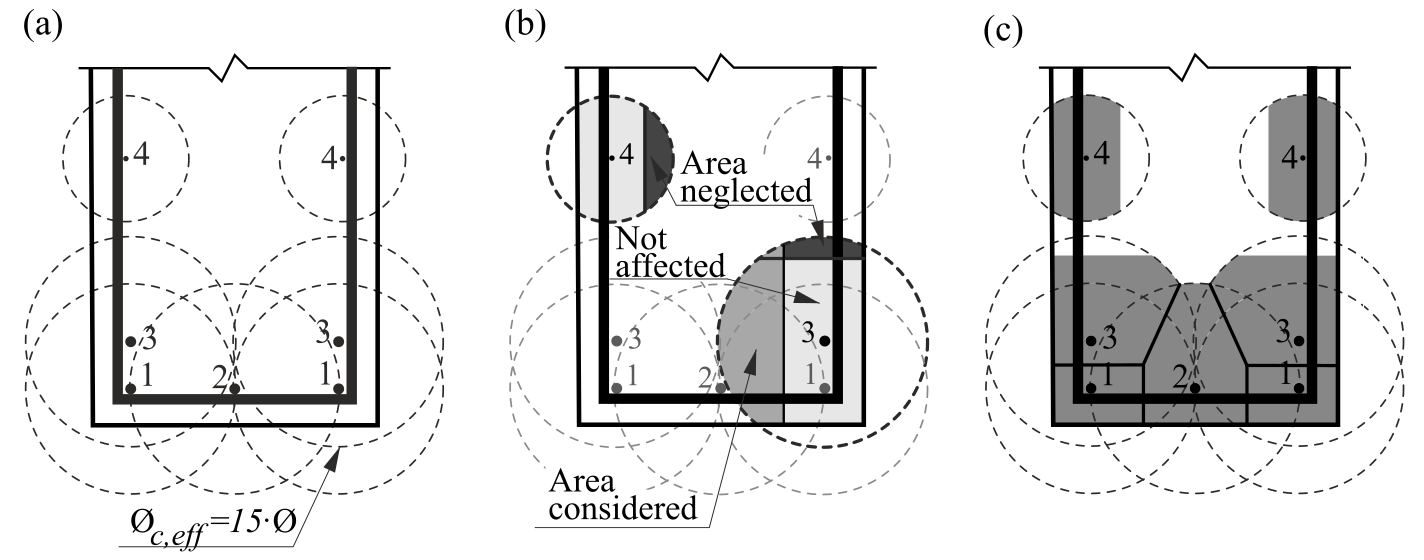$$\textsf{\textit{\footnotesize{Fig. 4\qquad Effective area of concrete in tension for stabilized cracking: (a) maximum concrete area that can be activated;}}}$$ $$\textsf{\textit{\footnotesize{(b) cover and global symmetry condition; (c) resultant effective area.}}}$$Non-stabilized crackingCracks existing in regions with geometric reinforcement ratios lower than ρcr, i.e., the minimum reinforcement amount for which the reinforcement is able to carry the cracking load without yielding, are generated by either non-mechanical actions (e.g. shrinkage) or the progression of cracks controlled by other reinforcement. The value of this minimum reinforcement is obtained as follows:${\rho _{cr}} = \frac{{{f_{ct}}}}{{{f_y} - \left( {n - 1} \right){f_{ct}}}}$where:fy reinforcement yield strength,fct concrete tensile strength,n modular ratio, n = Es / Ec .For conventional concrete and reinforcing steel, ρcr amounts to approximately 0.6%.For stirrups with reinforcement ratios below ρcr, cracking is considered to be non-stabilized and tension stiffening is implemented by means of the Pull-Out Model (POM) described in Fig. 3b. This model analyzes the behavior of a single crack considering no mechanical interaction between separate cracks, neglecting the deformability of concrete in tension and assuming the same stepped, rigid-perfectly plastic bond shear stress-slip relationship used by the TCM. This allows the reinforcement strain distribution (εs) in the vicinity of the crack to be obtained for any maximum steel stress at the crack (σsr) directly from equilibrium. Given the fact that the crack spacing is unknown for a non-fully developed crack pattern, the average strain (εm) is computed for any load level over the distance between points with zero slip when the reinforcing bar reaches its tensile strength (ft) at the crack (lε,avg in Fig. 3b), leading to the following relationships: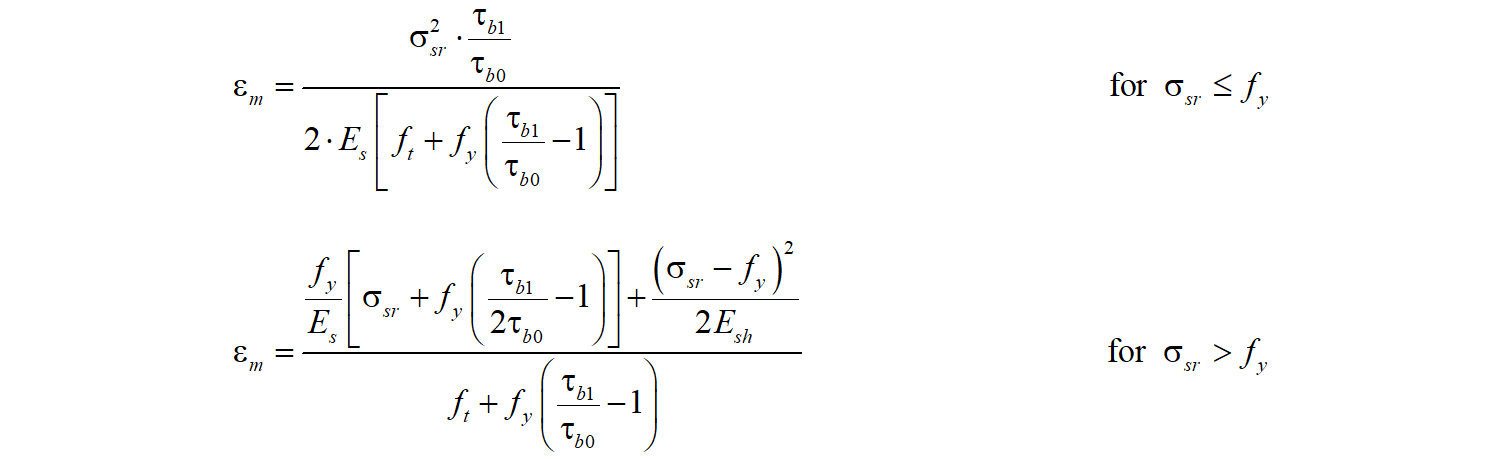The proposed models allow the computation of the behavior of bonded reinforcement, which is finally considered in the analysis. This behavior (including tension stiffening) for the most common European reinforcing steel (B500B, with ft / fy = 1.08 and εu = 5%) is illustrated in Fig. 3c-d. 2 Reinforcement design 2.1 Workflow and goalsThe goal of reinforcement design tools in the CSFM is to help designers determine the location and required amount of reinforcing bars efficiently. The following tools are available to help/ guide the user in this process: linear calculation, topology optimization and area optimization.Reinforcement design tools consider more simplified constitutive models than the models used for the final verification of the structure. Therefore, the definition of the reinforcement in this step should be considered a pre-design to be confirmed/refined during the final verification step. The use of the different reinforcement design tools will be depicted on the model shown in Fig. 5, which consists of one end of a simply supported beam with variable depth subjected to a uniformly distributed load.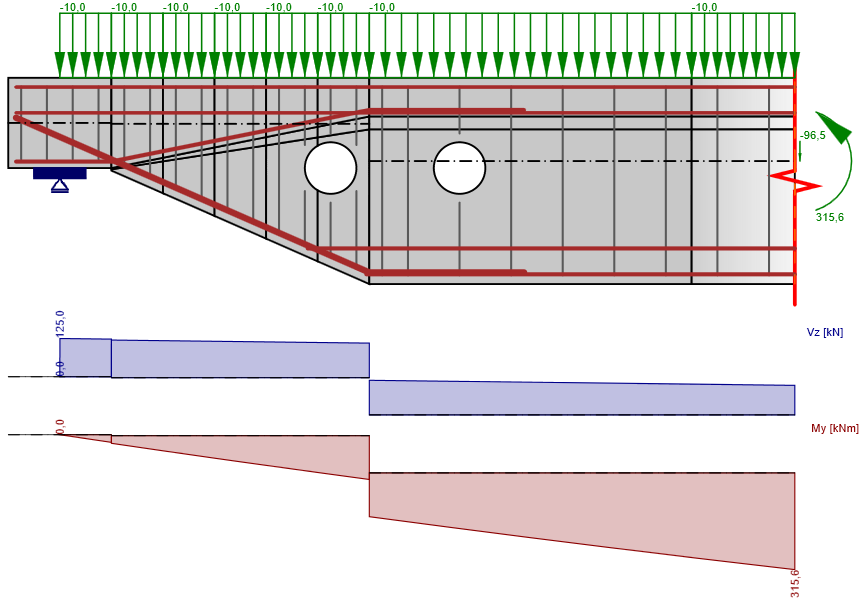$\textsf{\textit{\footnotesize{Fig. 5\qquad Model used to illustrate the use of the reinforcement design tools.}}}$2.2 Reinforcement locationsFor regions where the reinforcement layout is not known beforehand, there are two methods available in the CSFM to help the user determine the optimum location of reinforcing bars: linear analysis and topology optimization. Both tools provide an overview of the location of tensile forces in the uncracked region for a certain load case.2.2.1 Linear analysisLinear analysis considers linear elastic material properties and neglects reinforcement in the concrete region. It is, therefore, a very fast calculation that provides a first insight into the locations of tension and compression areas. An example of such a calculation is shown in Fig. 6.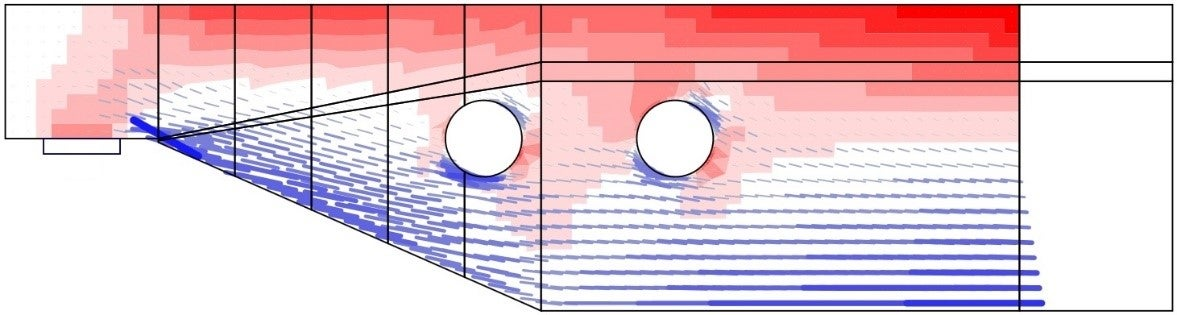$\textsf{\textit{\footnotesize{Fig. 6\qquad Results from the linear analysis tool for defining reinforcement layout}}}$$\textsf{\textit{\footnotesize{(red: areas in compression, blue: areas in tension).}}}$2.2.2 Topology optimizationTopology optimization is a method that aims to find the optimal distribution of material in a given volume for a certain load configuration. The topology optimization implemented in Idea StatiCa Detail uses a linear finite element model. Each finite element may have a relative density from 0 to 100 %, representing the relative amount of material used. These element densities are the optimization parameters in the optimization problem. The resulting material distribution is considered optimal for the given set of loads if it minimizes the total strain energy of the system. By definition, the optimal distribution is also the geometry that has the largest possible stiffness for the given loads.The iterative optimization process starts with a homogeneous density distribution. The calculation is performed for multiple total volume fractions (20%, 40%, 60% and 80%), which allows the user to select the most practical result, as proposed by . The resulting shape consists of trusses with struts and ties and represents the optimum shape for the given load cases (Fig. 7).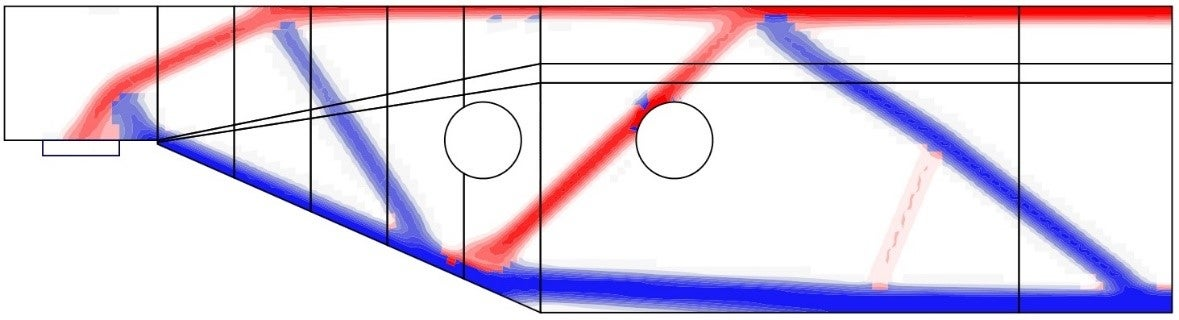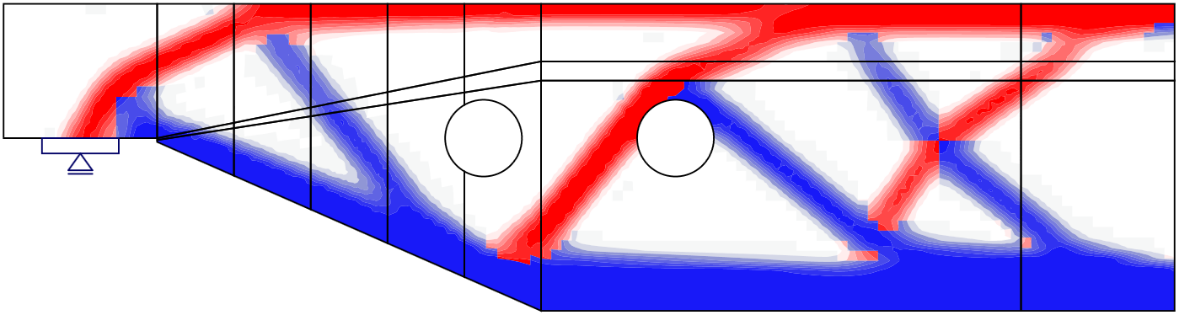$\textsf{\textit{\footnotesize{Fig. 7\qquad Results from the topology optimization design tool with 20\% and 40\% effective volume}}}$$\textsf{\textit{\footnotesize{(red: areas in compression, blue: areas in tension).}}}$ 3 Finite element implementation 3.1 IntroductionThe CSFM considers continuous stress fields in the concrete (2D finite elements), complemented by discrete “rod” elements representing the reinforcement (1D finite elements). Therefore, the reinforcement is not diffusely embedded into the concrete 2D finite elements, but explicitly modeled and connected to them. A plane stress state is considered in the calculation model.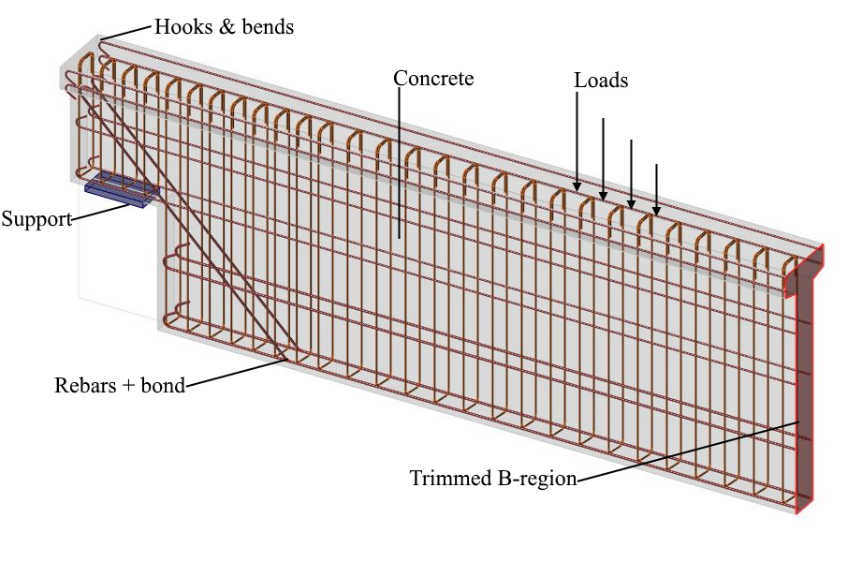$\textsf{\textit{\footnotesize{Fig. 8\qquad Visualization of the calculation model of a structural element (trimmed beam) in Idea StatiCa Detail.}}}$Both entire walls and beams, as well as details (parts) of beams (isolated discontinuity region, also called trimmed end), can be modeled. In the case of walls and entire beams, supports must be defined in such a way that an (externally) isostatic (statically determinate) or hyperstatic (statically indeterminate) structure results. The load transfer at the trimmed ends of beams is introduced by means of a special Saint-Venant transfer zone (described in Section 3.3), which ensures a realistic stress distribution in the analyzed detail region.3.2 Supports and load transmitting componentsTo model most of the situations during the construction process, many types of supports (Fig. 9) and components used for transferring load (Fig. 10) are available in the CSFM.3.2.1 SupportsPoint support can be modeled in several ways to ensure that stresses are not localized in one point but rather distributed over a larger area. The first option is a distributed point support (Fig. 9a), which uniformly distributes the load on the edge of member over the specified width.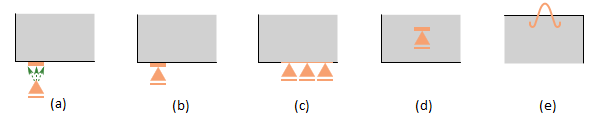$\textsf{\textit{\footnotesize{Fig. 9\qquad Various types of supports:}}}$$\textsf{\textit{\footnotesize{(a) point distributed; (b) bearing plate; (c) line support; (d) patch support; (e) hanging.}}}$Patch support (Fig. 9d), on the other hand, can only be placed inside a volume of concrete with a defined effective radius. It is then connected by rigid elements to the nodes of the reinforcement mesh within this radius. Therefore, it is required to define a reinforcing cage around patch support.For the more precise modeling of some real scenarios, there are two other options for point supports. Firstly, there is point support with a bearing plate of defined width and thickness (Fig. 9b). The material of the bearing plate can be specified, and the whole bearing plate is meshed independently. Secondly, there is hanging support available (Fig. 9e), which can be used for modeling lifting anchors or lifting studs.Line support (Fig. 9c) can be defined on an edge (by specifying its length) or inside an element (by a polyline). It is also possible to specify its stiffness and/or non-linear behavior (support in compression/tension or only in compression).3.2.2 Load transmitting componentsThe introduction of loads into the structure can also be modeled in several ways. For point loads, a bearing plate (Fig. 10a) can be used similarly as point support, distributing the concentrated load onto a larger area thanks to steel plate with defined width and thickness. Patch loads (Fig. 10b and Fig. 11) are placed inside the concrete with a certain effective radius and are connected by rigid elements to the nodes of nearby reinforcing bars.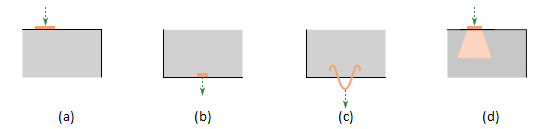$\textsf{\textit{\footnotesize{Fig. 10\qquad Various types of load transfer components:}}}$$\textsf{\textit{\footnotesize{(a) bearing plate; (b) patch load; (c) hanging; (d) partially loaded area.}}}$Lifting anchors or lifting studs can be modeled by a hanging load (Fig. 10c). User can use partially loaded area (Fig. 10d), which allows increasing load-bearing capacity of concrete in compression according to Eurocode (it is not possible to use this type of load transmitting component when ACI is set). The structure can also be loaded with line loads on the edges, by general polyline or by surface loads, representing, e.g., self-weight (which is not automatically considered in the analysis).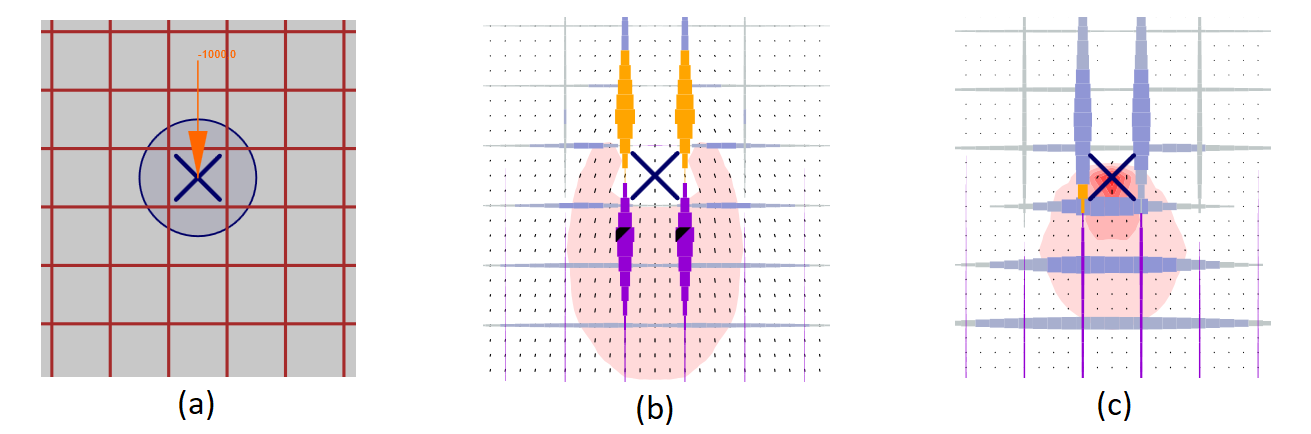$\textsf{\textit{\footnotesize{Fig. 11\qquad Patch load: (a) load application; (b) load transferred through rebars; (c) load transferred through concrete.}}}$3.3 Load transfer at trimmed ends of beamsIn many cases, we need to model only some detail (part) of a structural member such as beam support, opening in the middle of the beam, etc. This approach can lead to support configurations that are unstable but admissible in Idea StatiCa Detail (including the case of no supports). However, in such cases, it is also necessary to model the section representing the connection to the adjoining B-region, including internal forces at this section which satisfy the equilibrium. In certain cases (e.g., when modeling beam support), these internal forces can be determined automatically by the program.Between B-region and the analyzed discontinuity region, a Saint-Venant transfer zone is automatically created to ensure a realistic stress distribution in the analyzed region. The width of the transfer zone is determined as half of the section’s depth. As the only purpose of the Saint-Venant zone is to achieve a proper stress distribution in the rest of the model, no results from this area are displayed in verification, and no stop criteria are considered here.The edge of the Saint-Venant zone that represents the trimmed end of the beam is modeled as rigid, i.e., it may rotate, but must rest plane. This is done by connecting all the FEM nodes of the edge to a separate node at the center of inertia of the section using a rigid body element (RBE2). The internal forces of the element may then be applied at this node, as shown in Fig. 12.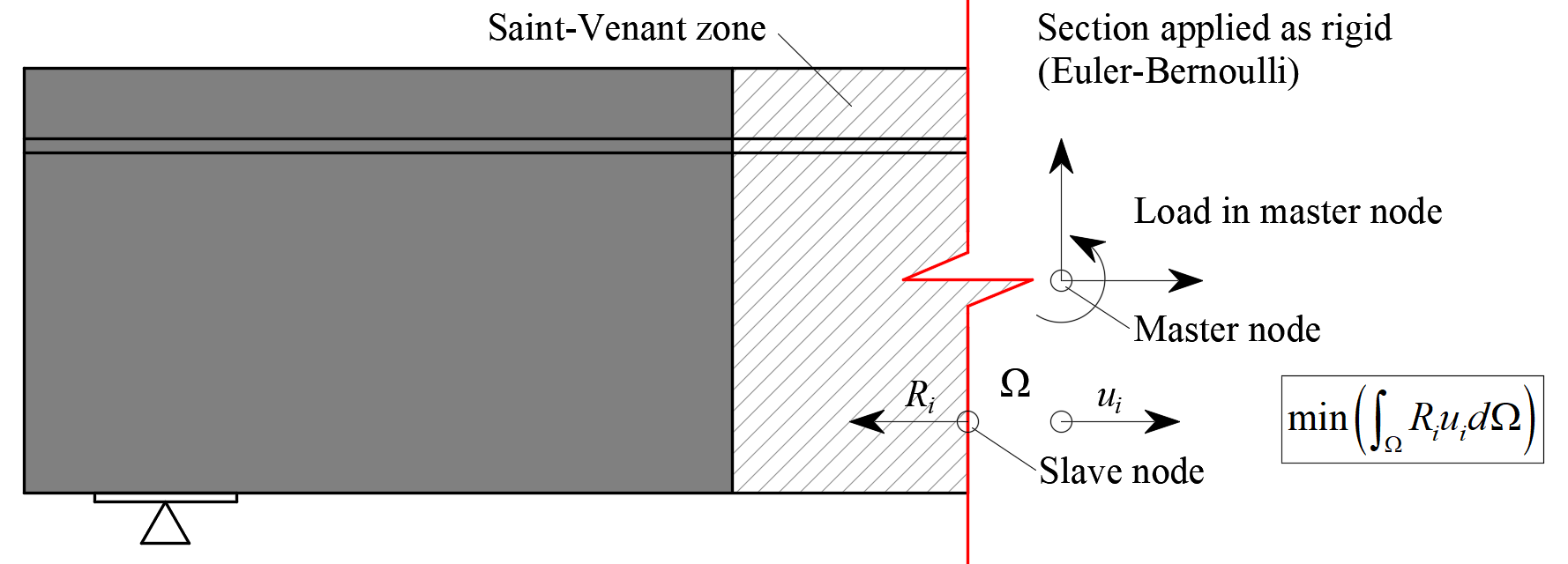$\textsf{\textit{\footnotesize{Fig. 12\qquad Transfer of internal forces at a trimmed end.}}}$3.4 Geometric modification of cross-sectionsIn the case of geometries which include haunches, the width of the wall elements used to model the haunch is reduced in comparison to the original width so that it is equal to double its height plus the thickness of the adjacent wall. This is based on the assumption that a compression stress field would expand from the wall at a 45° angle (see Fig. 13), so the aforementioned reduced width would be the maximal width capable of transferring loads.Note that the method of determining effective width flange implemented in CSFM is different from the one stated in 5.3.2.1 EN 1992-1-1 (2015). Besides geometry, Eurocode based effective width flange is explicitly affected by the span lengths and boundary conditions of a structure.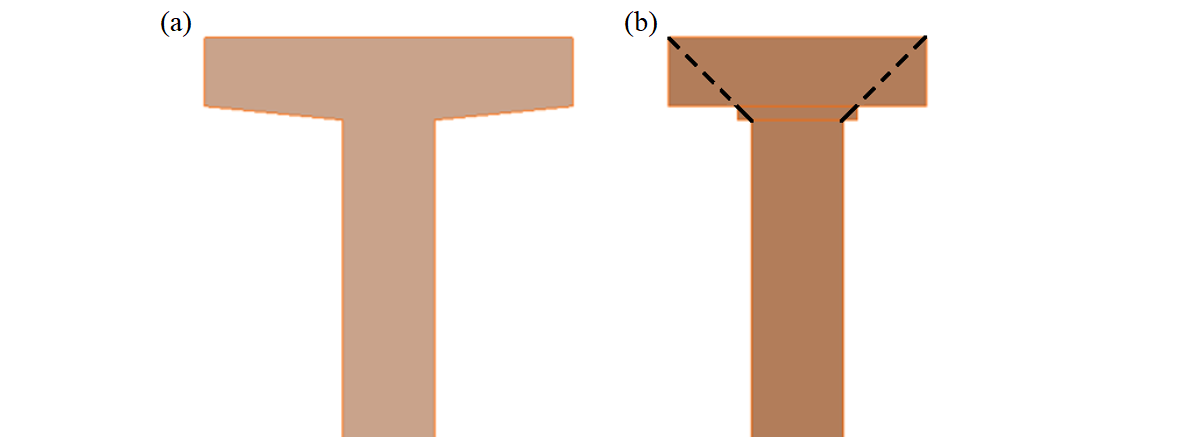$\textsf{\textit{\footnotesize{Fig. 13\qquad Width reduction of a cross-section: (a) user input; (b) FE model – automatically determined reduced flange width.}}}$In the case of haunches lying in the horizontal plane (Fig. 14), each haunch is divided into five sections along its length. Each of these sections is then modeled as a wall with a constant thickness, which is equal to the real thickness in the middle of the respective section.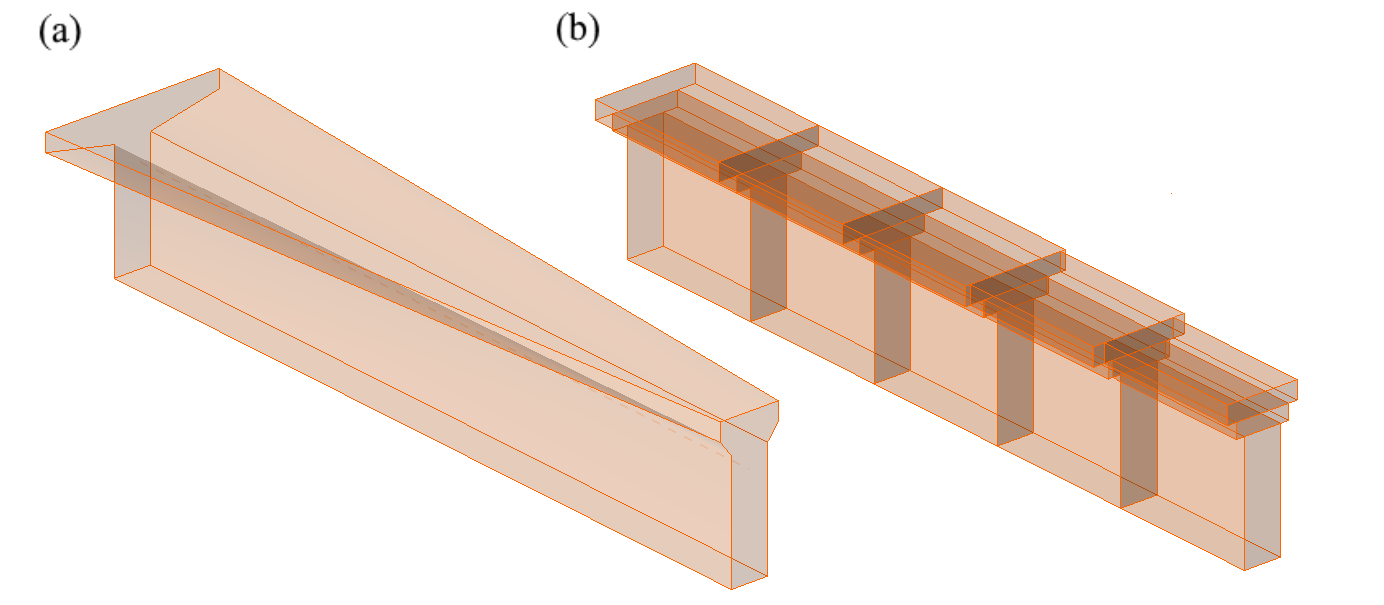$\textsf{\textit{\footnotesize{Fig. 14\qquad Horizontal haunch: (a) user input; (b) FE model – a haunch automatically divided into five sections.}}}$3.5 Finite element typesThe non-linear finite element analysis model is created by several types of finite elements used to model concrete, reinforcement, and the bond between them. Concrete and reinforcement elements are first meshed independently and then connected to each other using multi-point constraints (MPC elements). This allows the reinforcement to occupy an arbitrary, relative position in relation to the concrete. If anchorage length verification is to be calculated, bond and anchorage end spring elements are inserted between the reinforcement and the MPC elements.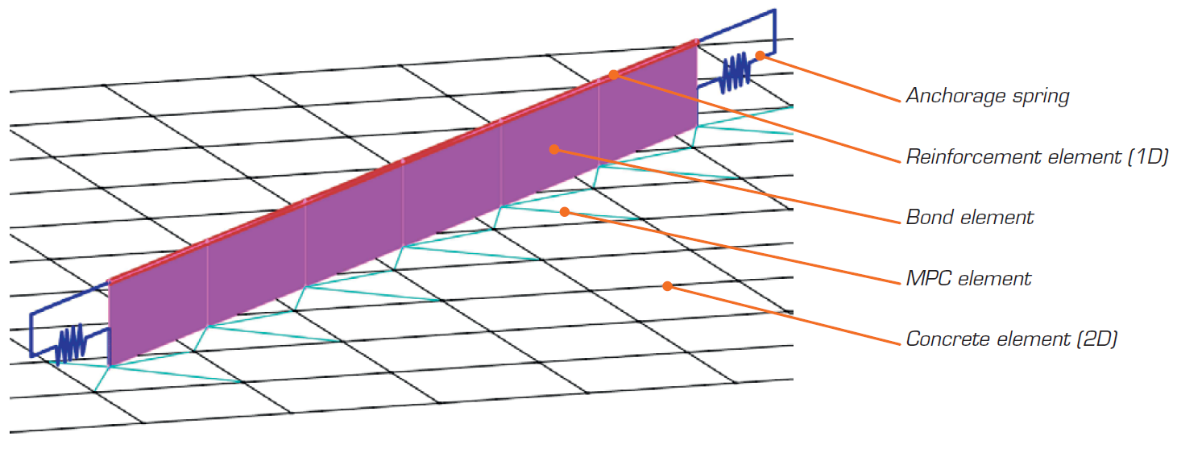$\textsf{\textit{\footnotesize{Fig. 15\qquad Finite element model: reinforcement elements mapped to concrete mesh using MPC elements and bond elements.}}}$3.5.1 ConcreteConcrete is modeled using quadrilateral and trilateral shell elements, CQUAD4 and CTRIA3. These can be defined by four or three nodes, respectively. Only plane stress is assumed to exist in these elements, i.e., stresses or strains in the z-direction are not considered.Each element has four or three integration points which are placed at approximately 1/4 of its size. At each integration point in every element, the directions of principal strains α1, α3 are calculated. In both of these directions, the principal stresses σc1, σc3 and stiffnesses E1, E2 are evaluated according to the specified concrete stress-strain diagram, as per Fig. 2. It should be noted that the impact of the compression softening effect couples the behavior of the main compressive direction to the actual state of the other principal direction.3.5.2 ReinforcementRebars are modeled by two-node 1D “rod” elements (CROD), which only have axial stiffness. These elements are connected to special “bond” elements which were developed in order to model the slip behavior between a reinforcing bar and the surrounding concrete. These bond elements are subsequently connected by MPC (multi-point constraint) elements to the mesh representing the concrete. This approach allows the independent meshing of reinforcement and concrete, while their interconnection is ensured later.3.5.3 Anchorage length verification: bond elementsThe anchorage length is verified by implementing the bond shear stresses between concrete elements (2D) and reinforcing bar elements (1D) in the finite element model. To this end, a “bond” finite element type was developed.The definition of the bond element is similar to that of a shell element (CQUAD4). It is also defined by 4 nodes, but in contrast to a shell, it only has a non-zero stiffness in shear between the two upper and two lower nodes. In the model, the upper nodes are connected to the elements representing reinforcement and the lower nodes to those representing concrete. The behavior of this element is described by the bond stress, τb, as a bilinear function of the slip between the upper and lower nodes, δu, see Fig. 16.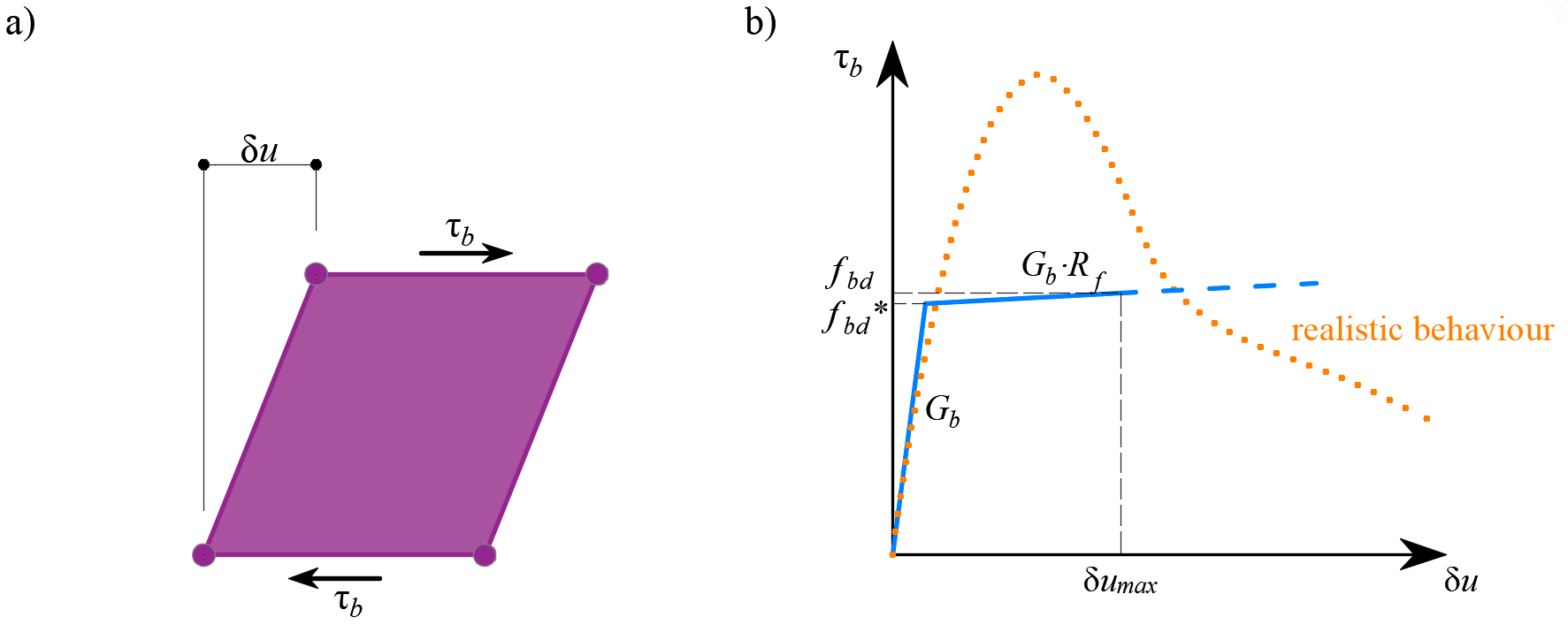$\textsf{\textit{\footnotesize{Fig. 16\qquad (a) conceptual illustration of the deformation of a bond element; (b) a stress-deformation function.}}}$The elastic stiffness modulus of the bond-slip relationship, Gb, is defined as follows:$G_b = k_g \cdot \frac{E_c}{Ø}$where:kg coefficient depending on the reinforcing bar surface (by default kg = 0.2),Ec modulus of elasticity of concrete, taken as EcmØ the diameter of the reinforcing bar.The design values of ultimate bond shear stress, fbd, provided in the respective selected design codes EN 1992-1-1 (2015) or ACI 318-04 are used to verify the anchorage length. The hardening of the plastic branch is calculated by default as Gb/105.3.5.4 Anchorage length verification: spring elementsThe provision of anchorage ends to the reinforcing bars (i.e., bends, hooks, loops…), which fulfills the prescriptions of design codes, allows the reduction of the basic anchorage length of the bars (lb,net) by a certain factor β (referred to as the ‘anchorage coefficient’ below). The design value of the anchorage length (lb) is then calculated as follows:$l_b = \left(1 - \beta\right)l_{b,net}$The available anchorage types in the CSFM include a straight bar (i.e., no anchor end reduction), bend, hook, loop, welded transverse bar, perfect bond and continuous bar. All these types, along with the respective anchorage coefficients β, are shown in Fig. 17 for longitudinal reinforcement and in Fig. 18 for stirrups. The values of the adopted anchorage coefficients are in accordance with EN 1992-1-1. It should be noted that in spite of the different available options, the CSFM just distinguishes three types of anchorage ends: (i) no reduction in the anchorage length, (ii) a reduction of 30 % of the anchorage length in the case of a normalized anchorage and (iii) perfect bond.$\textsf{\textit{\footnotesize{Fig. 17\qquad Available anchorage types and respective anchorage coefficients for longitudinal reinforcing bars in the CSFM:}}}$$\textsf{\textit{\footnotesize{(a) straight bar; (b) bend; (c) hook; (d) loop; (e) welded transverse bar; (f) perfect bond; (g) continuous bar.}}}$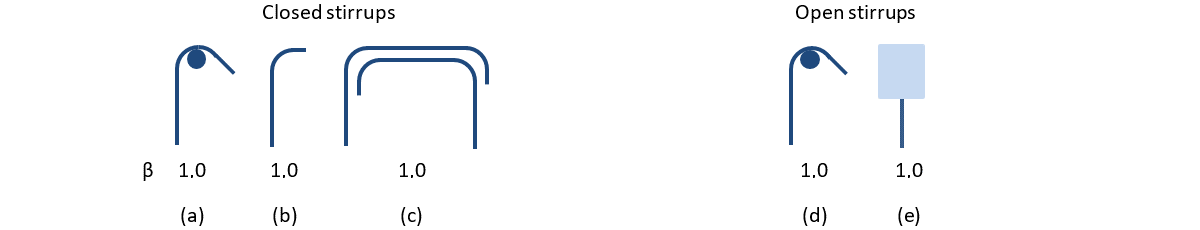$\textsf{\textit{\footnotesize{Fig. 18\qquad Available anchorage types and respective anchorage coefficients for stirrups.}}}$$\textsf{\textit{\footnotesize{Closed stirrups: (a) hook; (b) bend; (c) overlap. Open stirrups: (d) hook; (e) continuous bar.}}}$The intended reduction in lb,net is equivalent to the activation of the reinforcing bar at its end at a percentage of its maximum capacity given by the anchorage reduction coefficient, as shown in Fig. 19a.$\textsf{\textit{\footnotesize{Fig. 19\qquad Model for the reduction of the anchorage length:}}}$$\textsf{\textit{\footnotesize{(a) anchorage force along the anchorage length of the reinforcing bar; (b) slip-anchorage force constitutive relationship.}}}$The reduction of the anchorage length is included in the finite element model by means of a spring element at the end of the bar (Fig. 15), which is defined by the constitutive model shown in Fig. 19b. The maximum force transmitted by this spring (Fau) is:$F_{au} = \beta \cdot A_s \cdot f_{yd}$where :β the anchorage coefficient based on anchorage type,As the cross-section of the reinforcing bar,fyd the design value of the yield strength of the reinforcement.3.6 MeshingThe finite elements described in the previous chapter are implemented internally, and the analysis model is generated automatically without any need for proficient user interaction. An important part of this process is meshing.3.6.1 ConcreteAll concrete members are meshed together. A recommended element size is automatically computed by the application based on the size and shape of the structure and taking into account the diameter of the largest reinforcing bar. Moreover, the recommended element size guarantees that a minimum of 4 elements are generated in thin parts of the structure, such as slim columns or thin slabs, to ensure reliable results in these areas. The maximum number of concrete elements is limited to 5000, but this value is sufficient to provide the recommended element size for most structures. Designers can always select a user-defined concrete element size by modifying the multiplier of the default mesh size.3.6.2 ReinforcementThe reinforcement is divided into elements with approximately the same length as the concrete element size. Once the reinforcement and concrete meshes are generated, they are interconnected with bond elements (ULS) or directly by MPC elements (SLS), as shown in Fig. 15.3.6.3 Bearing platesAuxiliary structural parts, such as bearing plates, are meshed independently. The size of these elements is calculated as 2/3 of the size of concrete elements in the connection area. The nodes of the bearing plate mesh are then connected to the edge nodes of the concrete mesh using interpolation constraint elements (RBE3).3.6.4 Loads and supportsPatch loads and patch supports are connected only to the reinforcement, as shown in Fig. 20. Therefore, it is necessary to define the reinforcement around them. Connection to all nodes of the reinforcement within the effective radius is ensured by RBE3 elements with equal weight.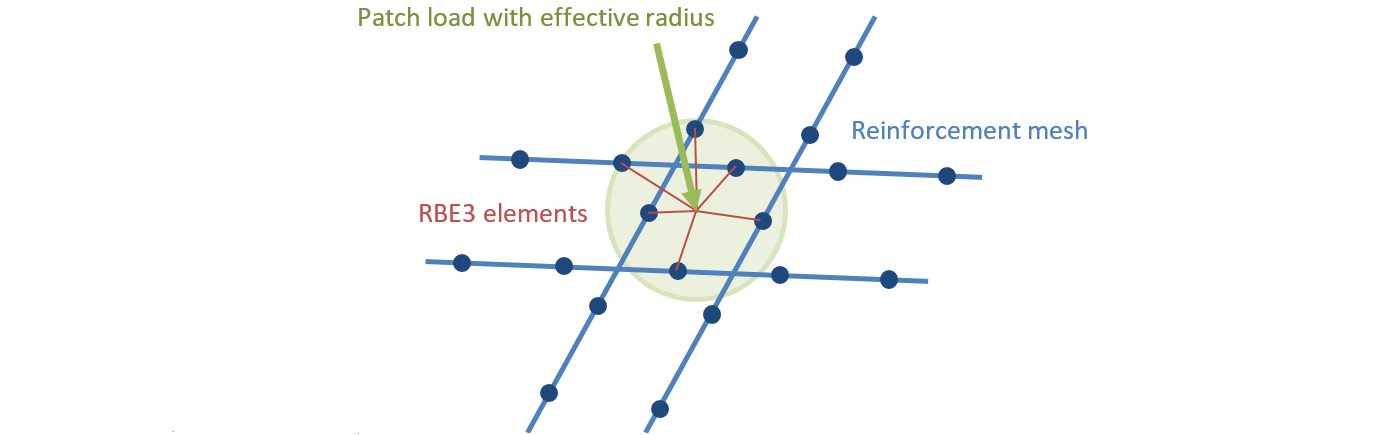$\textsf{\textit{\footnotesize{Fig. 20\qquad Patch load mapping to reinforcement mesh.}}}$Line supports and line loads are connected to the nodes of the concrete mesh using RBE3 elements based on the specified width or effective radius. The weight of the connections is inversely proportional to the distance from the support or load impulse.3.7 Solution method and load-control algorithmA standard full Newton-Raphson (NR) algorithm is used to find the solution to a non-linear FEM problem. The implementation is almost identical to the one presented in .Generally, the NR algorithm does not often converge when the full load is applied in a single step. A usual approach, which is also used here, is to apply the load sequentially in multiple increments and use the result from the previous load increment to start the Newton solution of a subsequent one. For this purpose, a load control algorithm was implemented on top of the Newton-Raphson. In the case that the NR iterations do not converge, the current load increment is reduced to half its value and the NR iterations are retried.A second purpose of the load-control algorithm is to find the critical load, which corresponds to certain “stop criteria” – specifically the maximum strain in concrete, the maximum slip in bond elements, the maximum displacement in anchorage elements and the maximum strain in reinforcing bars. The critical load is found using the bisection method. In the case that the stop criterion is exceeded anywhere in the model, the results of the last load increment are discarded, and a new increment of half the size of the previous one is calculated. This process is repeated until the critical load is found with a certain error tolerance.For concrete, the stop criterion was set by default to a 5% strain in compression (i.e., around an order of magnitude larger than the actual failure strain of concrete) and 7% in tension at the integration points of shell elements. In tension, the value was set to allow for the limit strain in reinforcement, which is usually around 5% without accounting for tension stiffening, to be reached first. In compression, the value was chosen from among several alternatives, as one that is large enough for the effects of crushing to be visible in the results, but small enough so as not to cause too many problems with numerical stability.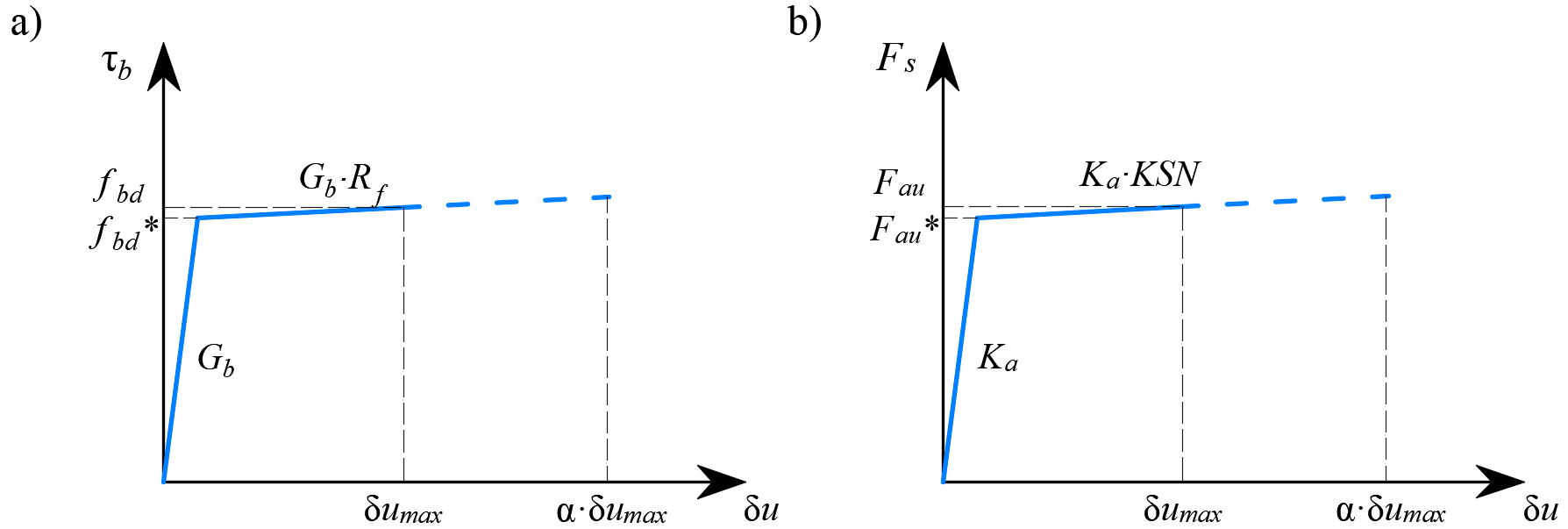$\textsf{\textit{\footnotesize{Fig. 21\qquad Constitutive relationship of bond and anchorage elements used for anchorage length verification:}}}$$\textsf{\textit{\footnotesize{(a) bond shear stress slip response of a bond element; (b) force-displacement response of an anchorage element.}}}$For reinforcement, the stop criterion is defined in terms of stresses. Since stresses at the crack are modeled, the criterion in tension corresponds to the reinforcement tensile strength accounting for the safety coefficient. The same value is used for the criterion in compression.The stop criterion in bond and anchorage elements is α·δumax, where δumax is the maximal slip used in code checks and α = 10.3.8 Presentation of resultsResults are presented independently for concrete and for reinforcement elements. The stress and strain values in concrete are calculated at the integration points of shell elements. However, as it is not practical to present the data in such a manner, the results are presented by default in nodes, like the maximal value of compressive stress from adjacent gauss integration points in connected elements (Fig. 22). It should be noted that this representation might locally underestimate the results at compressed edges of members in a case that the finite-element size is similar to the depth of the compression zone.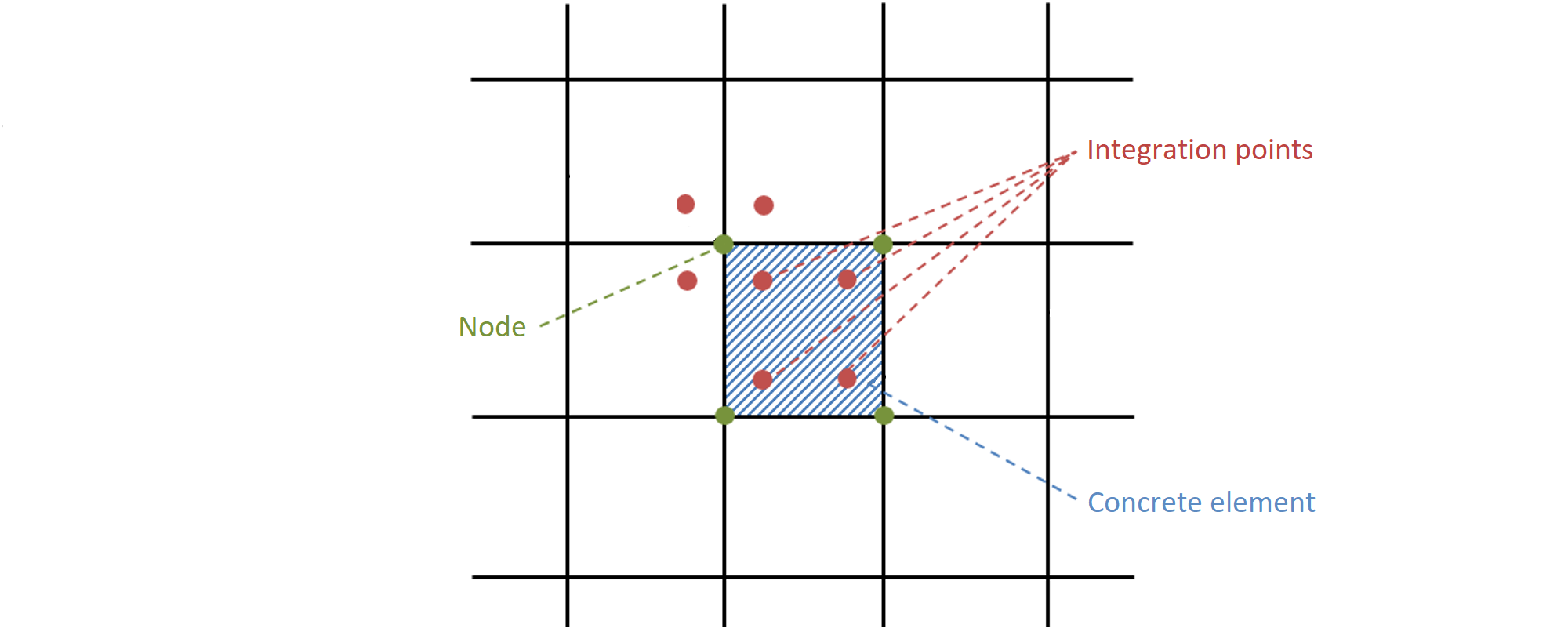$\textsf{\textit{\footnotesize{Fig. 22\qquad Concrete finite element with integration points and nodes: presentation of the results for concrete in nodes and}}}$$\textsf{\textit{\footnotesize{in finite elements.}}}$The results for the reinforcement finite elements are either constant for each element (one value – e.g., for steel stresses) or linear (two values – for bond results). For auxiliary elements, such as elements of bearing plates, only deformations are presented. 4 Verification of the structural element Assessment of the structure using the CSFM is performed by two different analyses: one for serviceability, and one for ultimate limit state load combinations. The serviceability analysis assumes that the ultimate behavior of the element is satisfactory, and the yield conditions of the material will not be reached at serviceability load levels. This approach enables the use of simplified constitutive models (with a linear branch of concrete stress-strain diagram) for serviceability analysis to enhance numerical stability and calculation speed. Therefore, it is recommended the use of the workflow presented below, in which the ultimate limit state analysis is carried out as the first step.4.1 Ultimate limit state analysisThe different verifications required by specific design codes are assessed based on the direct results provided by the model. ULS verifications are carried out for concrete strength, reinforcement strength, and anchorage (bond shear stresses).To ensure a structural element has an efficient design, it is highly recommended to run a preliminary analysis which takes into account the following steps: Choose a selection of the most critical load combinations. Calculate only Ultimate Limit State (ULS) load combinations. Use a coarse mesh (by increasing the multiplier of the default mesh size in Setup (Fig. 23)).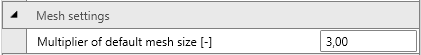$\textsf{\textit{\footnotesize{Fig. 23\qquad Mesh multiplier.}}}$Such a model will calculate very quickly, allowing designers to review the detailing of the structural element efficiently and re-run the analysis until all verification requirements are fulfilled for the most critical load combinations. Once all the verification requirements of this preliminary analysis are fulfilled, it is suggested that the complete ultimate load combinations be included, and the use of a fine mesh size (the mesh size recommended by the program). User can change mesh size by the multiplier, which can reach value from 0.5 to 5 (Fig. 23).The basic results and verifications (stress, strain and utilization (i.e., the calculated value/limit value from the code), as well as the direction of principal stresses in the case of concrete elements) are displayed by means of different plots where compression is generally presented in red and tension in blue. Global minimum and maximum values for the entire structure can be highlighted as well as minimum and maximum values for every user-defined part. In a separate tab of the program, advanced results such as tensor values, deformations of the structure and reinforcement ratios (effective and geometric) used for computing the tension stiffening of reinforcing bars can be shown. Furthermore, loads and reactions for selected combinations or load cases can be presented.4.2 Serviceability limit state analysisSLS assessments are carried out for stress limitation, crack width and deflection limits. Stresses are checked in concrete and reinforcement elements according to the applicable code in a similar manner to that specified for the ULS.The serviceability analysis contains certain simplifications of the constitutive models which are used for ultimate limit state analysis. A perfect bond is assumed, i.e., the anchorage length is not verified at serviceability. Furthermore, the plastic branch of the stress-strain curve of concrete in compression is disregarded, while the elastic branch is linear and infinite. These simplifications enhance the numerical stability and calculation speed, and do not reduce the generality of the solution as long as the resultant material stress limits at serviceability are clearly below their yielding points (as required by standards). Therefore, the simplified models used for serviceability are only valid if all verification requirements are fulfilled.4.2.1 Crack width calculationThere are two ways of computing crack widths - stabilized and non-stabilized cracking. According to the geometrical reinforcement ratio in each part of the structure is decided, which type of crack calculation model will be used (TCM for stabilized cracking and POM for non-stabilized cracking model).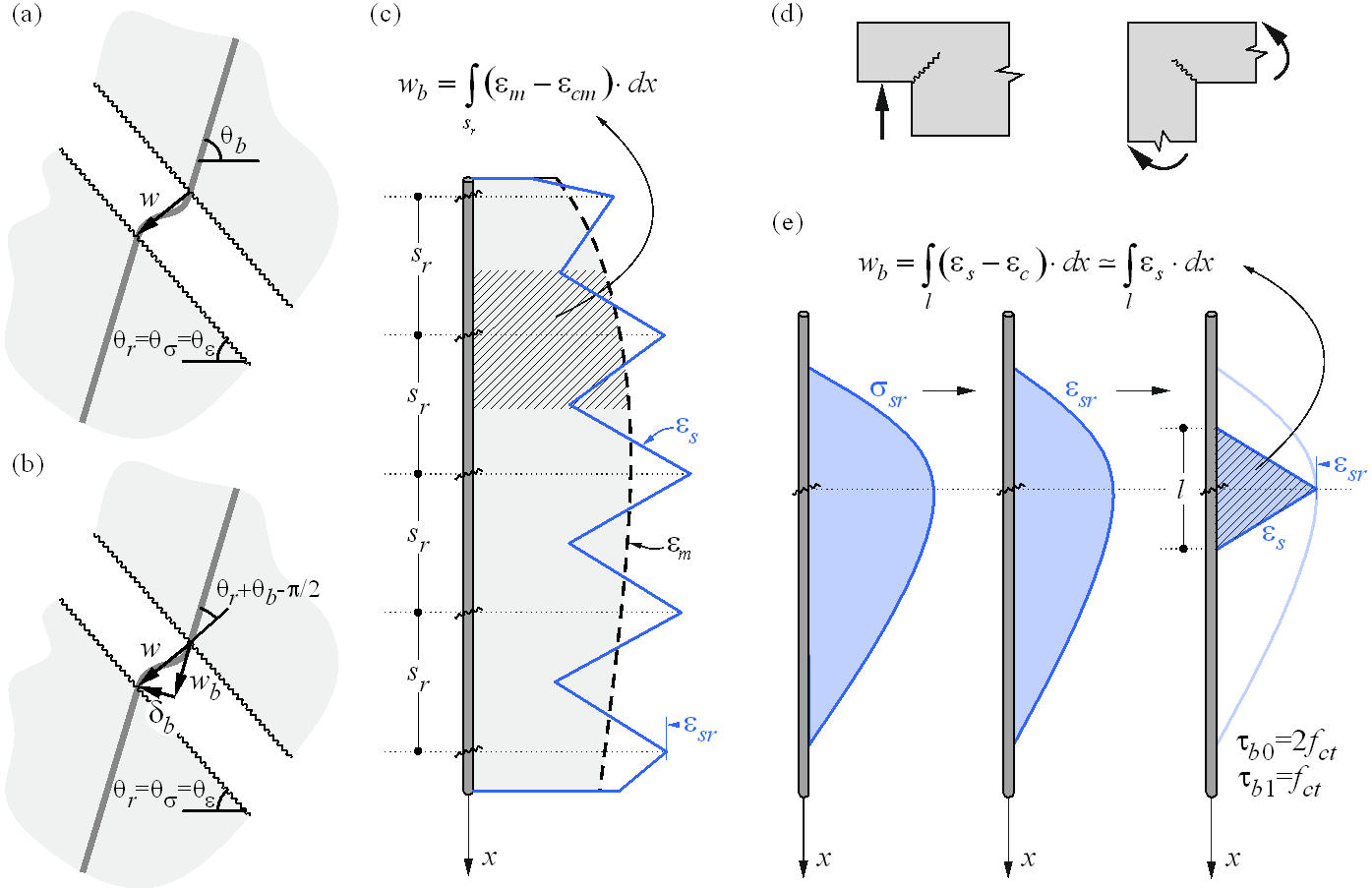$$\textsf{\textit{\footnotesize{Fig. 24 \qquad Crack width calculation: (a) considered crack kinematics; (b) projection of crack kinematics into the principal}}}$$ $$\textsf{\textit{\footnotesize{directions of the reinforcing bar; (c) crack width in the direction of the reinforcing bar for stabilized cracking; (d) cases with}}}$$ $$\textsf{\textit{\footnotesize{local non-stabilized cracking regardless of the reinforcement amount; (e) crack width in the direction of the reinforcing bar}}}$$$$\textsf{\textit{\footnotesize{for non-stabilized cracking.}}}$$While the CSFM yields a direct result for most verifications (e.g., member capacity, deflections…), crack width results are calculated from the reinforcement strain results directly provided by FE analysis following the methodology described in Fig. 24. A crack kinematic without slip (pure crack opening) is considered (Fig. 24a), which is consistent with the main assumptions of the model. The principal directions of stresses and strains define the inclination of the cracks (θr = θs= θe). According to (Fig. 24b), the crack width (w) can be projected in the direction of the reinforcing bar (wb), leading to:$w = \frac{w_b}{\cos\left(θ_r + θ_b - \frac{π}{2}\right)}$where θb is the bar inclination.The component wb is consistently calculated based on the tension stiffening models presented in Section 1.2.4 by integrating the reinforcement strains. For those regions with fully developed crack patterns, the calculated average strains (em) along the reinforcing bars are directly integrated along the crack spacing (sr), as indicated in (Fig. 24c). While this approach to calculating the crack directions does not correspond to the real position of the cracks, it still provides representative values that lead to crack width results that can be compared to code-required crack width values at the position of the reinforcing bar.Special situations are observed at concave corners of the calculated structure. In this case, the corner predefines the position of a single crack that behaves in a non-stabilized fashion before additional adjacent cracks develop. These additional cracks generally develop after the serviceability range (Mata-Falcón 2015), which justifies calculating the crack widths in such a region as if they were non-stabilized (Fig. 25) by means of the model presented in Section 1.2.4.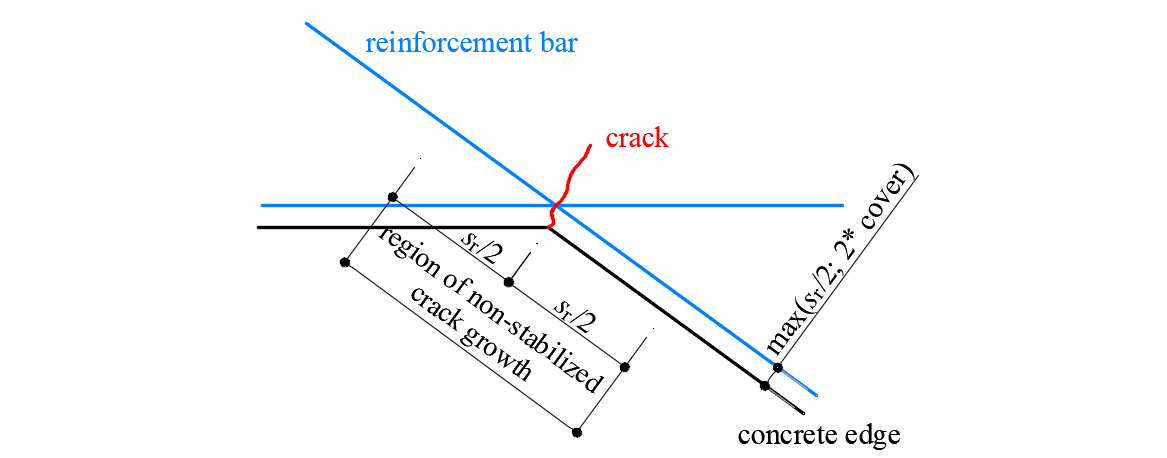$\textsf{\textit{\footnotesize{Fig. 25\qquad Definition of the region at concave corners in which the crack width is computed as if it were non-stabilized.}}}$ 5 Verification of the structural elements according to Eurocode Assessment of the structure using the CSFM is performed by two different analyses: one for serviceability, and one for ultimate limit state load combinations. The serviceability analysis assumes that the ultimate behavior of the element is satisfactory, and the yield conditions of the material will not be reached at serviceability load levels. This approach enables the use of simplified constitutive models (with a linear branch of concrete stress-strain diagram) for serviceability analysis to enhance numerical stability and calculation speed.5.1 Materials5.1.1 Concrete - ULSThe concrete model implemented in the CSFM is based on the uniaxial compression constitutive laws prescribed by EN 1992-1-1 for the design of cross-sections, which only depend on compressive strength. The parabola-rectangle diagram specified in EN 1992-1-1 (Fig. 26a) is used by default in the CSFM, but designers can also choose a more simplified elastic ideal plastic relationship (Fig. 26b). The tensile strength is neglected, as it is in classic reinforced concrete design.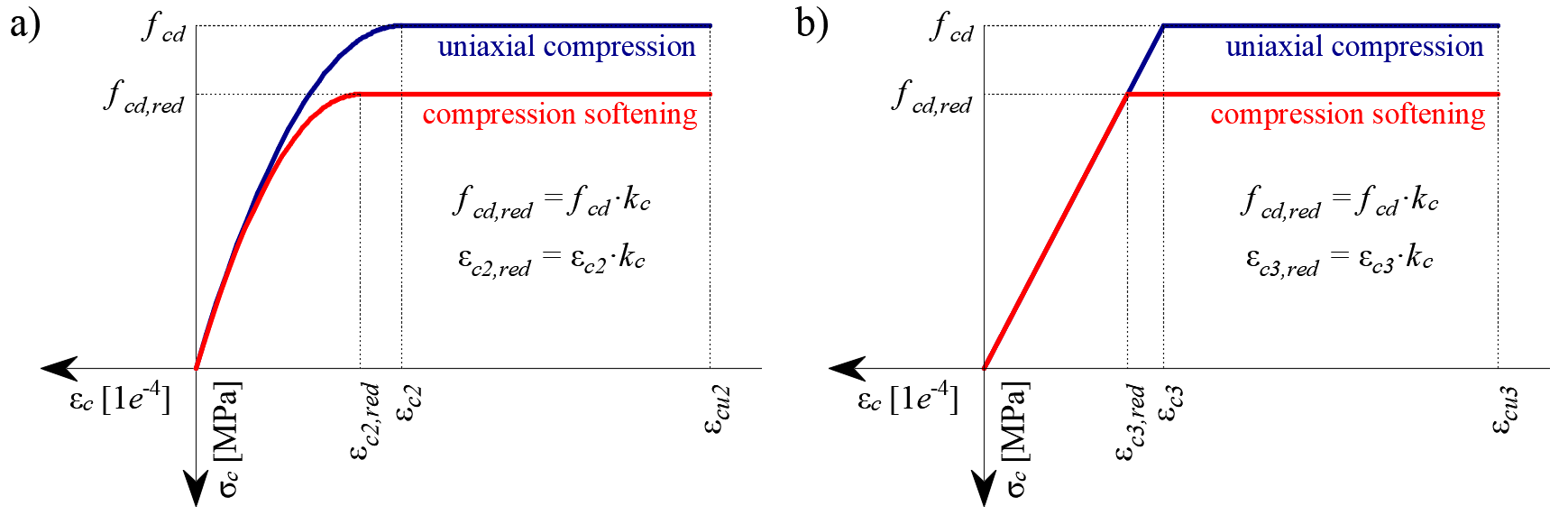$\textsf{\textit{\footnotesize{Fig. 26\qquad The stress-strain diagrams of concrete for ULS: a) parabola-rectangle diagram; b) bilinear diagram.}}}$Using default settings, the current implementation of the CSFM in IDEA StatiCa Detail does not consider an explicit failure criterion in terms of strains for concrete in compression (i.e., after the peak stress is reached it considers a plastic branch with εcu2 (εcu3) in value 5% while EN 1992-1-1 assumes ultimate strain less than 0,35%). This simplification does not allow the deformation capacity of structures failing in compression to be verified. However, their ultimate capacity is properly predicted when, in addition to the factor of cracked concrete (kc2 defined in (Fig. 27)), the increase in the brittleness of concrete as its strength rises is considered by means of the $$\eta_{fc}$$ reduction factor defined in fib Model Code 2010 as follows:$f_{cd}=\frac{f_{ck,red}}{γ_c} = \frac{k_c \cdot f_{ck}}{γ_c} = \frac{\eta _{fc} \cdot k_{c2} \cdot f_{ck}}{γ_c}$${\eta _{fc}} = {\left( {\frac{{30}}{{{f_{ck}}}}} \right)^{\frac{1}{3}}} \le 1$where:kc is the global reduction factor of the compressive strengthkc2 is the reduction factor due to the presence of transverse crackingfck is the concrete cylinder characteristic strength (in MPa for the definition of $$\eta_{fc}$$).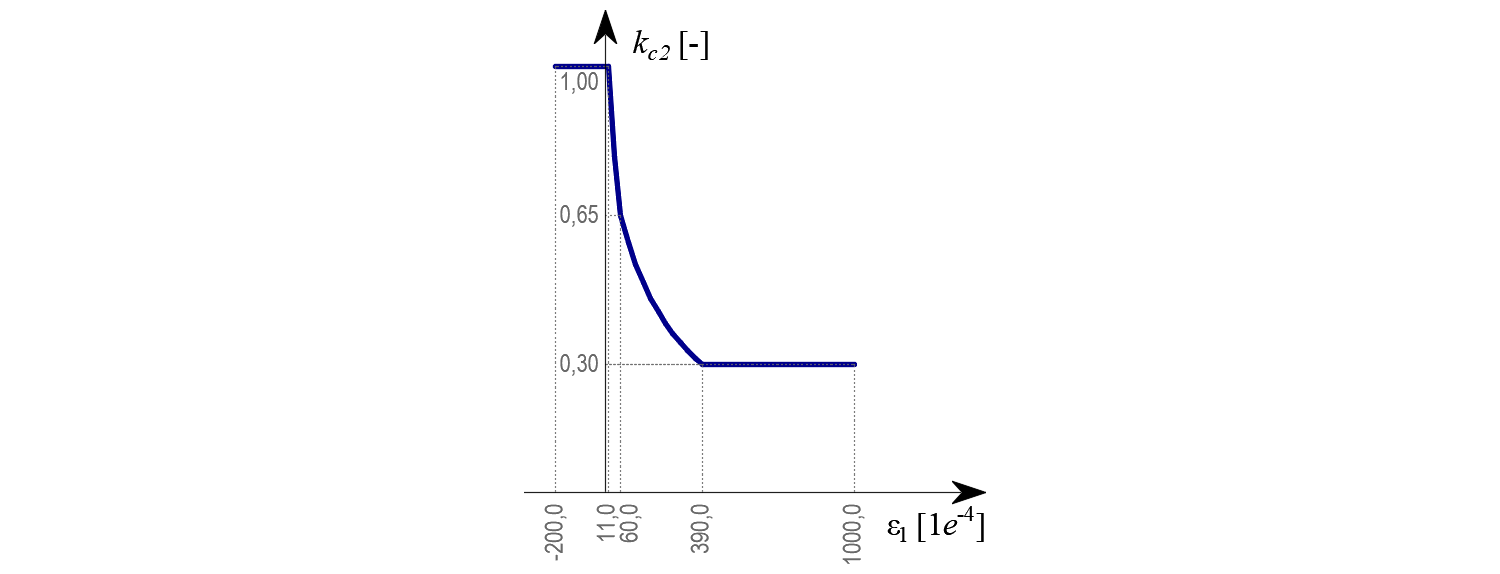$\textsf{\textit{\footnotesize{Fig. 27\qquad The compression softening law.}}}$5.1.2 Concrete - SLSThe serviceability analysis contains certain simplifications of the constitutive models which are used for ultimate limit state analysis. A perfect bond is assumed, i.e., the anchorage length is not verified at serviceability. Furthermore, the plastic branch of the stress-strain curve of concrete in compression is disregarded, while the elastic branch is linear and infinite. Compression softening law is not considered. These simplifications enhance the numerical stability and calculation speed and do not reduce the generality of the solution as long as the resultant material stress limits at serviceability are clearly below their yielding points (as required by Eurocode). Therefore, the simplified models used for serviceability are only valid if all verification requirements are fulfilled.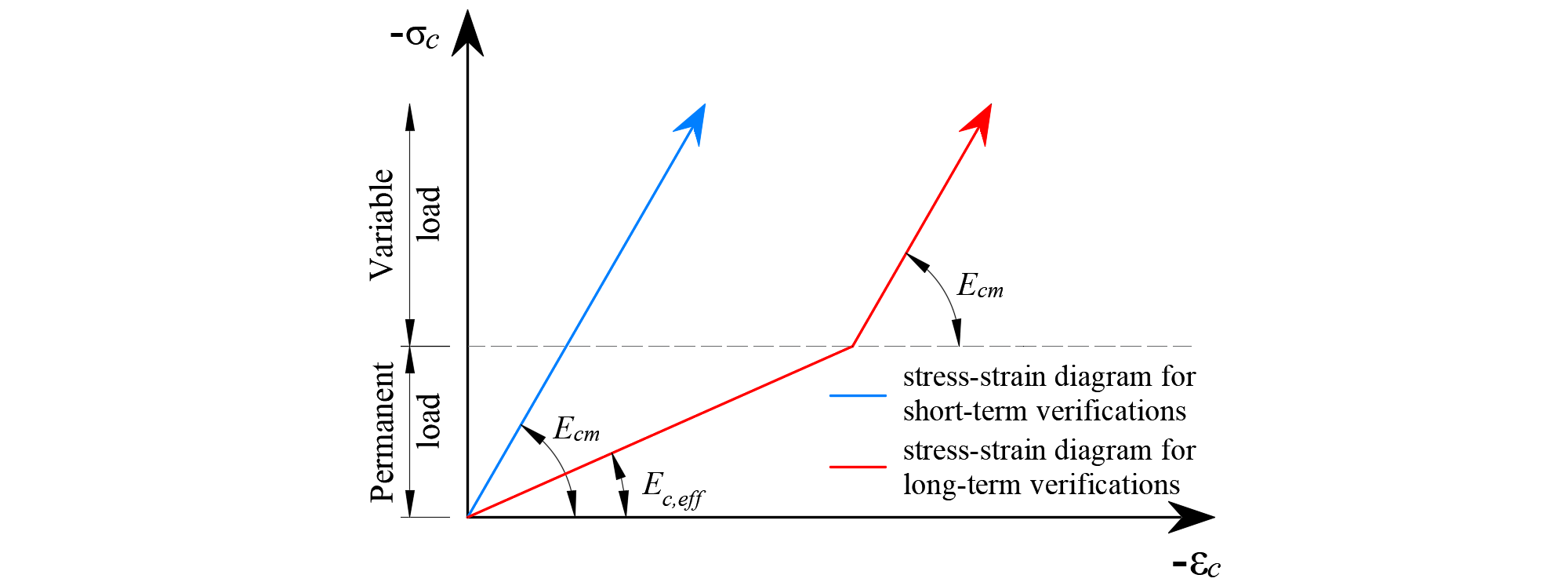$\textsf{\textit{\footnotesize{Fig. 28\qquad Concrete stress-strain diagrams implemented for serviceability analysis: short- and long-term verifications.}}}$Long term effectsIn serviceability analysis, the long-term effects of concrete are considered using an effective infinite creep coefficient ($$\varphi$$, taken as a value of 2.5 by default) which modifies the secant modulus of elasticity of concrete (Ecm) as follows:$E_{c,eff} = \frac{E_{cm}}{1+\varphi}$When considering long-term effects, a load step with all permanent loads is first calculated considering the creep coefficient (i.e., using the effective modulus of elasticity of concrete, Ec,eff) and, then, the additional loads are calculated without the creep coefficient (i.e., using Ecm). In addition, to conduct short-term verifications, another calculation is performed in which all loads are calculated without the creep coefficient. Both calculations for long and short-term verifications are depicted in Fig. 28.5.1.3 ReinforcementBy default, the idealized bilinear stress-strain diagram for the bare reinforcing bars typically defined by design codes (Fig. 29) is considered. The definition of this diagram only requires basic properties of the reinforcement to be known during the design phase (strength and ductility class). Whenever known, the actual stress-strain relationship of the reinforcement (hot-rolled, cold-worked, quenched and self-tempered, …) can be considered. The reinforcement stress-strain diagram can be defined by the user, but in this case, it is impossible to assume tension stiffening effect (it is impossible to calculate crack width). Using the stress-strain diagram with horizontal top branch does not allow to the verification of structural durability. Therefore, manual verification of standard ductility requirements is necessary.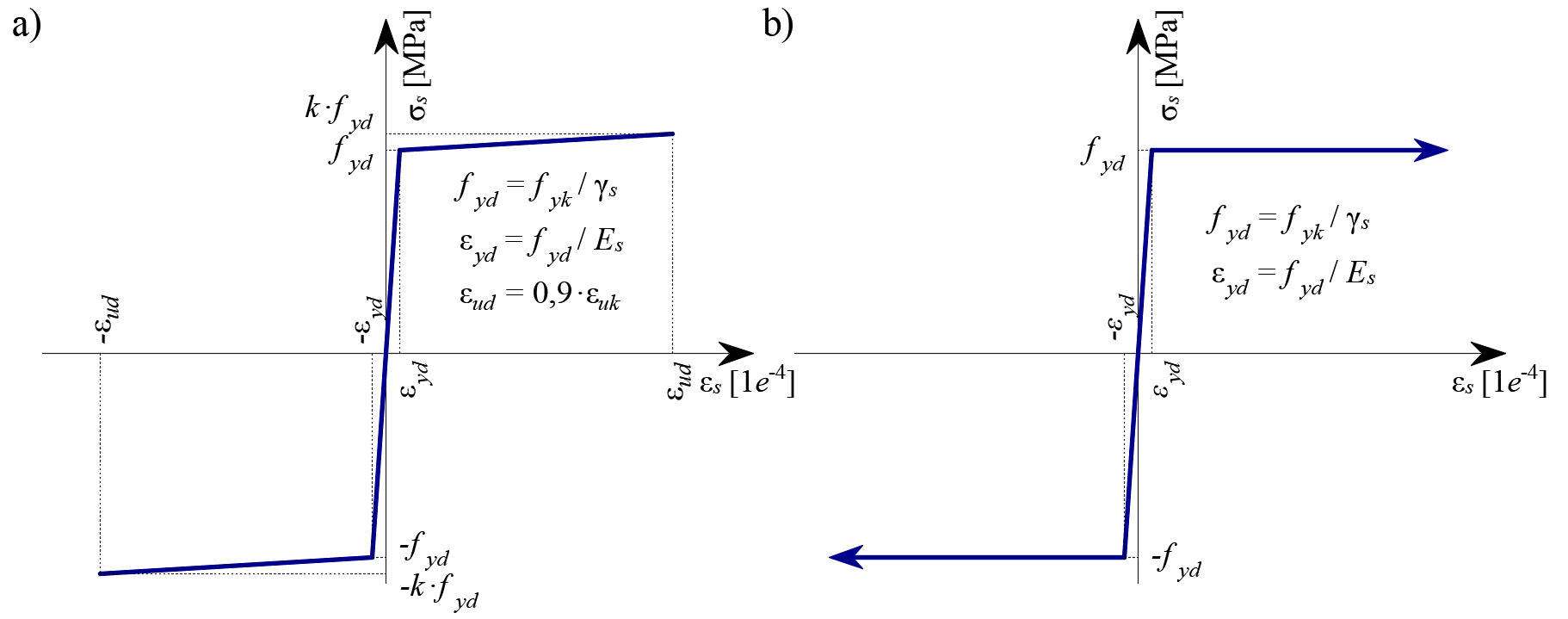$$\textsf{\textit{\footnotesize{Fig. 29 \qquad Stress-strain diagram of reinforcement: a) bilinear diagram with an inclined top branch; b) bilinear diagram}}}$$ $$\textsf{\textit{\footnotesize{with a horizontal top branch.}}}$$Tension stiffening (Fig. 30) is accounted for automatically by modifying the input stress-strain relationship of the bare reinforcing bar in order to capture the average stiffness of the bars embedded in the concrete (εm), following the approaches presented in Section 1.2.4.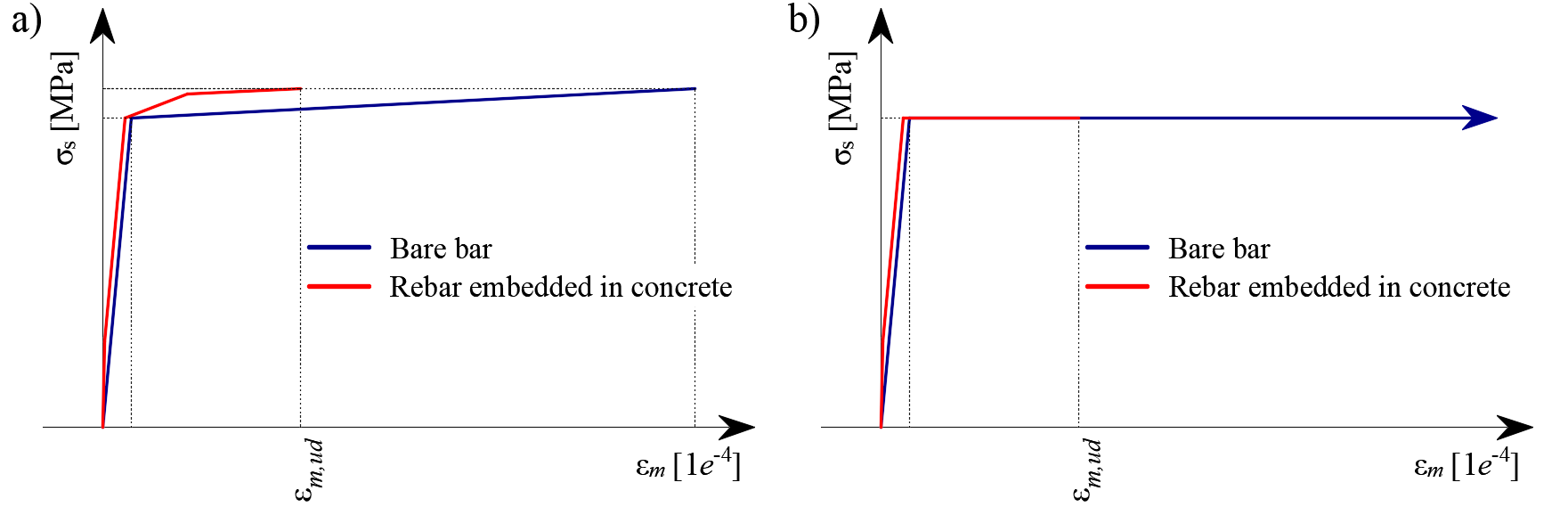$\textsf{\textit{\footnotesize{Fig. 30\qquad Scheme of tension stiffening.}}}$5.2 Safety format factorThe Compatible Stress Field Method is compliant with modern design codes. As the calculation models only use standard material properties, the partial safety factor format prescribed in the design codes can be applied without any adaptation. In this way, the input loads are factored and the characteristic material properties are reduced using the respective safety coefficients prescribed in design codes, exactly as in conventional concrete analysis. Values of material safety factors prescribed in EN 1992-1-1 chap. 2.4.2.4 are set by default, but user can change safety factors in Setup (Fig. 31).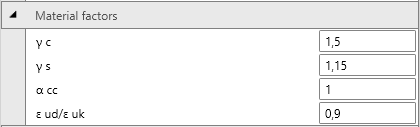$\textsf{\textit{\footnotesize{Fig. 31\qquad The setting of material safety factors in Idea StatiCa Detail.}}}$Load safety factors has to be defined by user in Combination rule for each non-linear combination of load cases (Fig. 32). For all templates implemented in Idea StatiCa Detail, partial safety factors are already predefined.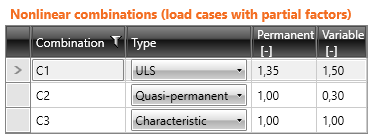$\textsf{\textit{\footnotesize{Fig. 32\qquad The setting of load partial factors in Idea StatiCa Detail.}}}$By using appropriate user-defined combinations of partial safety factors, users can also compute with the CSFM using the global resistance factor method (Navrátil, et al. 2017), but this approach is hardly ever used in design practice. Some guidelines recommend using the global resistance factor method for non-linear analysis. However, in simplified non-linear analyses (such as the CSFM), which only require those material properties that are used in conventional hand calculations, it is still more desirable to use the partial safety format.5.3 Ultimate limit state analysisThe different verifications required by EN 1992-1-1 are assessed based on the direct results provided by the model. ULS verifications are carried out for concrete strength, reinforcement strength, and anchorage (bond shear stresses).The concrete strength in compression is evaluated as the ratio between the maximum principal compressive stress σc3 obtained from FE analysis and the limit value σc3,lim. Then:$\frac{σ_{c3}}{σ_{c3,lim}}$$σ_{c3,lim} = f_{cd} = α_{cc} \cdot \frac{f_{ck,red}}{γ_c} = α_{cc} \cdot \frac{k_c \cdot f_{ck}}{γ_c} = α_{cc} \cdot \frac{\eta _{fc} \cdot k_{c2} \cdot f_{ck}}{γ_c}$${\eta _{fc}} = {\left( {\frac{{30}}{{{f_{ck}}}}} \right)^{\frac{1}{3}}} \le 1$where:fck characteristic cylinder strength of concrete,kc2 compression softening factor (see 5.1.1),γc is the partial safety factor for concrete, γc = 1,5,αcc is the coefficient taking account of long term effects on the compressive strength and of unfavourable effects resulting from the way the load is applied. The default value is 1,0. The strength of the reinforcement is evaluated in both tension and compression as the ratio between the stress in the reinforcement at the cracks σsr and the specified limit value σsr,lim:$\frac{σ_{sr}}{σ_{sr,lim}}$$$σ_{c3,lim} = \frac{k \cdot f_{yk}}{γ_s}\qquad\qquad\textsf{\small{for bilinear diagram with inclined top branch}}$$$$σ_{c3,lim} = \frac{f_{yk}}{γ_s}\qquad\qquad\,\,\,\,\textsf{\small{for bilinear diagram with horizontal top branch}}$$where:fyk yield strength of the reinforcement,k the ratio of tensile strength ftk to the yield stress, $$k = \frac{f_{tk}}{f_{yk}}$$γs is the partial safety factor for reinforcement γs = 1,15.The bond shear stress is evaluated independently as the ratio between the bond stress τb calculated by FE analysis and the ultimate bond strength fbd, according to EN 1992-1-1 chap. 8.4.2:$\frac{τ_{b}}{f_{bd}}$$f_{bd} = 2.25 \cdot η_1\cdot η_2\cdot f_{ctd}$where: fctd is the design value of concrete tensile strength. Due to the increasing brittleness of higher strength concrete, fctk,0,05 is limited to the value for C60/75, η1 is a coefficient related to the quality of the bond condition and the position of the bar during concreting (Fig. 33): η1 = 1,0 when ‘good’ conditions are obtained and η1 = 0,7 for all other cases and for bars in structural elements built with slip-forms, unless it can be shown that ‘good’ bond conditions existη2 is related to the bar diameter: η2 = 1,0 for Ø ≤ 32 mm η2 = (132 - Ø)/100 for Ø > 32 mm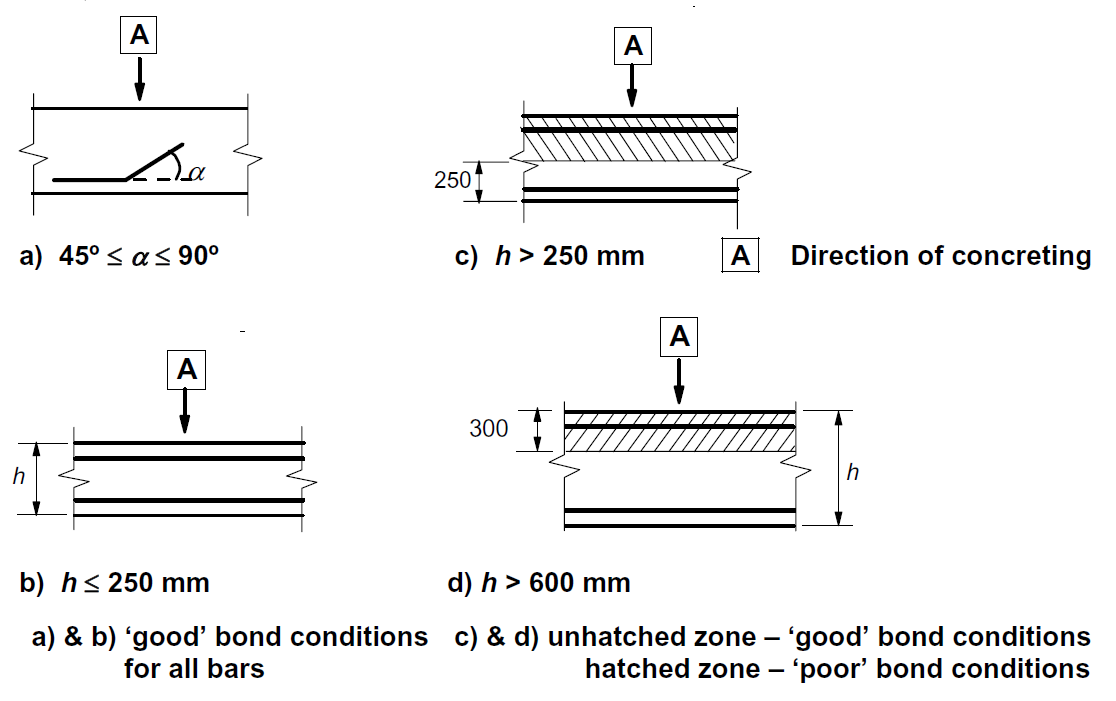$\textsf{\textit{\footnotesize{Fig. 33\qquad Description of bond conditions.}}}$These verifications are carried out with respect to the appropriate limit values for the respective parts of the structure (i.e., in spite of having a single grade both for concrete and reinforcement material, the final stress-strain diagrams will differ in each part of the structure due to tension stiffening and compression softening effects).5.4 Partially loaded areasWhen designing concrete structures, we meet two large groups of partially loaded areas (PLA) - the first of these comprises bearings, while the other consists of anchoring areas. According to currently valid standards for the design of reinforced concrete structures EN 1992-1-1 chap. 6.7 (Fig. 34), local crushing of concrete and transverse tension forces should be considered for partially loaded areas. For a uniformly distributed load on an area, Ac0, the compressive capacity of concrete may be increased by up to three times depending on the design distribution area, Ac1 (according to the new Eurocode concept, it is possible to increase load-bearing capacity up to seven times).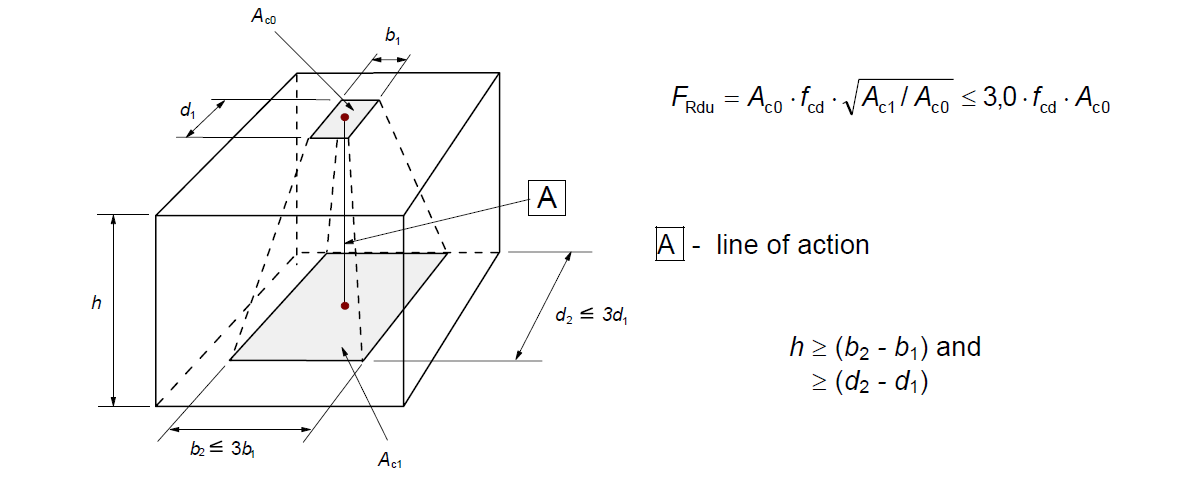$\textsf{\textit{\footnotesize{Fig. 34\qquad Partially loaded areas according to EN 1992-1-1.}}}$The partially loaded area must be sufficiently reinforced with transverse reinforcement designed to transmit the bursting forces that occur in the area. For the design of transverse reinforcement in partially loaded areas, the Strut-and-Tie method is used according to the Eurocode. Without the required transverse reinforcement, it is not possible to consider increasing the compressive capacity of the concrete.Partially loaded areas in the CSFM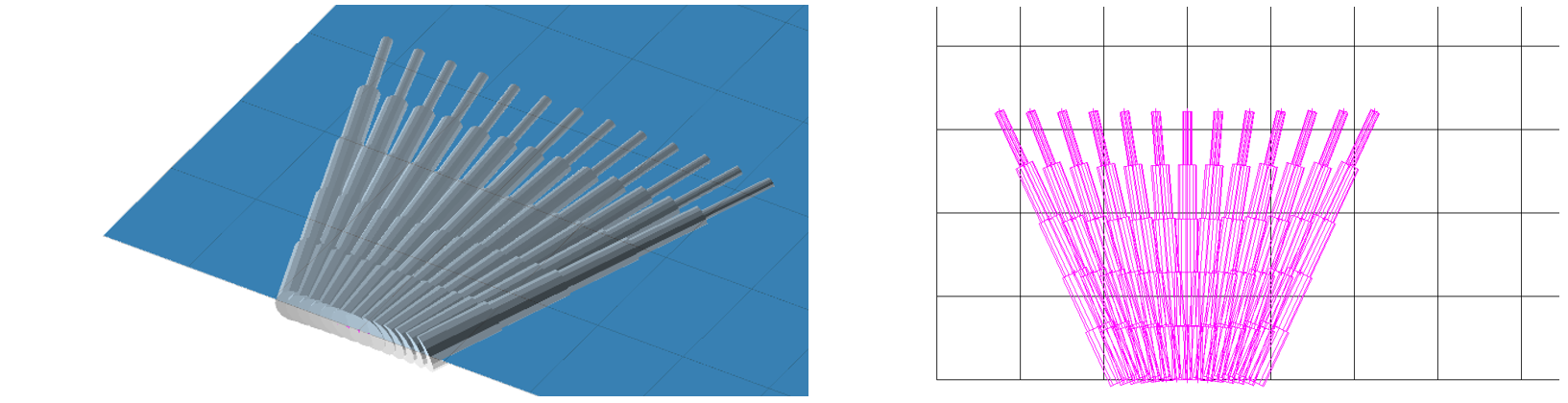$\textsf{\textit{\footnotesize{Fig. 35\qquad Fictitious struts with concrete finite element mesh.}}}$Using the CSFM, it is possible to design and assess reinforced concrete structures while including the influence of the increasing compressive resistance of concrete in partially loaded areas. Because the CSFM is a wall (2D) model and the partially loaded areas are a spatial (3D) task, it was necessary to find a solution that combines these two different types of tasks (Fig. 35). If the “partially loaded areas” function is activated, the allowable cone geometry is created according to the Eurocode (Fig. 34). All geometric collisions are solved fully in 3D for the specified concrete member geometry and the dimensions of each PLA. Subsequently, a computational model of the partially loaded area is created.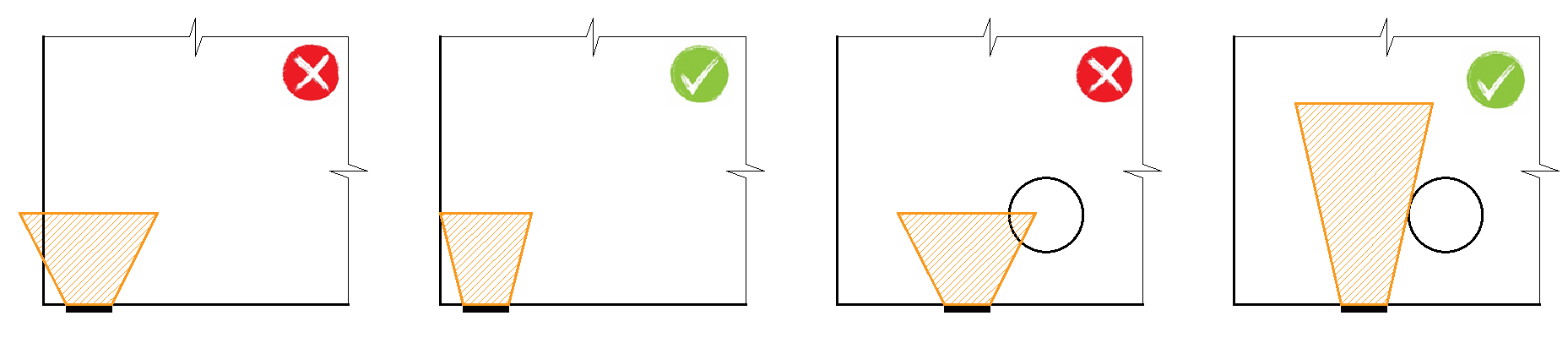$\textsf{\textit{\footnotesize{Fig. 36\qquad Allowable cone geometries.}}}$The modification of the material model proved to be an unsuitable approach, which was mainly because the mapping of properties to the finite element mesh is problematic. It was determined that an approach independent of the finite element mesh is a more appropriate solution. Absolutely coherent fictitious struts are created for the known compression cone geometry (Fig. 35 and Fig. 37). These struts have identical material properties to the concrete used in the model, including the stress-strain diagram. The shape of the cone determines the direction of the struts, which gradually distributes the load over the PLA to the design distribution area. The area density of the fictitious struts is variable at each part of the cone, and it adds a fictitious concrete area in the load direction. At the level of the loaded area (Ac0), a fictitious area of concrete is added according to the ratio $$\sqrt{A_{c0} \cdot A_{c1}} - A_{real}$$ (where Areal is an area of the support assumed in the 2D computational model), and this area decreases linearly to zero towards the design distribution area (Ac1). This solution ensures that the compressive stress in the concrete is constant over the entire cone volume.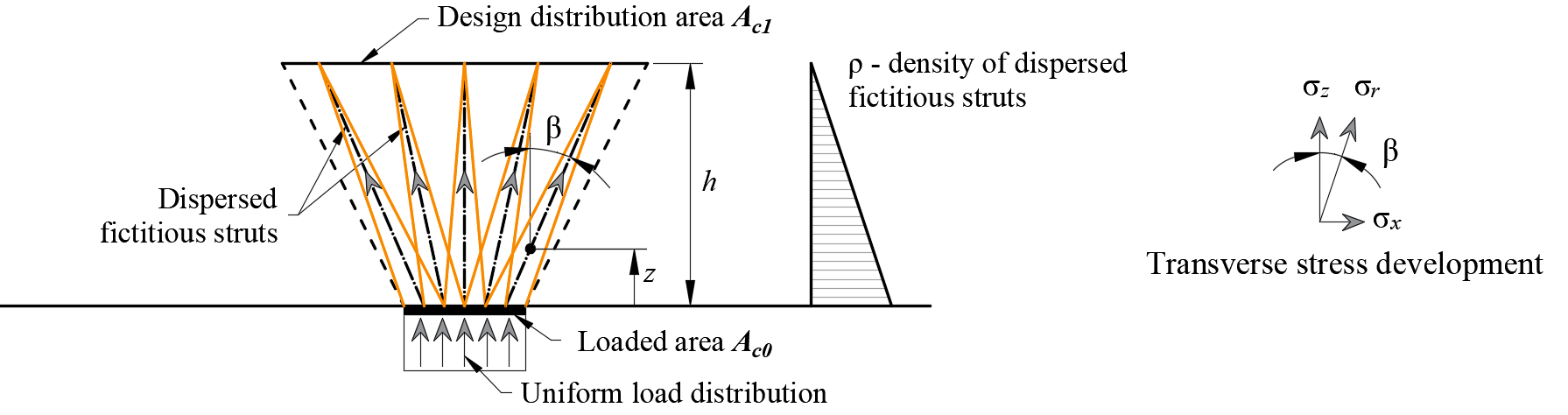$\rho \left( {\beta ,z} \right) = \left( {\sqrt {\frac{A_{c1}}{A_{c0}}} - \frac{A_{real}}{A_{c0}}} \right)\,\cdot\,\left( {1 - \frac{z}{h}} \right)\,\cdot\,\frac{1}{{\cos \beta }}$$\textsf{\textit{\footnotesize{Fig. 37\qquad Fictitious struts in the computational model}}}$The resistance of the partially loaded area is increased according to the ratio of the design distributed area and the loaded area laid in EN 1992-1-1 (6.7). It should be remembered that this is a design model that cannot precisely describe the stress state over a partially loaded area whose actual flow is much more complicated. However, this solution allows the correct distribution of load to the whole model while respecting the increased load capacity of the partially loaded area. In addition, it correctly introduces transverse stresses in this area.5.5 Serviceability limit state analysisSLS assessments are carried out for stress limitation, crack width and deflection limits. Stresses are checked in concrete and reinforcement elements according to EN 1992-1-1 in a similar manner to that specified for the ULS.5.5.1 Stress limitationThe compressive stress in the concrete shall be limited in order to avoid longitudinal cracks. According to EN 1992-1-1 chap. 7.2 (2), longitudinal cracks may occur if the stress level under the characteristic combination of loads exceeds a value k1fck. The concrete stress in compression is evaluated as the ratio between the maximum principal compressive stress σc3 obtained from FE analysis for serviceability limit states and the limit value σc3,lim. Then:$\frac{σ_{c3}}{σ_{c3,lim}}$$σ_{c3,lim} = k_1\cdot f_{ck}$where:fck characteristic cylinder strength of concrete,k1 =0.6.Unacceptable cracking or deformation may be assumed to be avoided if, under the characteristic combination of loads, the tensile stress in the reinforcement does not exceed k3fyk (EN 1992-1-1 chap. 7.2 (5)). The strength of the reinforcement is evaluated as the ratio between the stress in the reinforcement at the cracks σsr and the specified limit value σsr,lim:$\frac{σ_{sr}}{σ_{sr,lim}}$$σ_{sr,lim} = k_3\cdot f_{yk}$where:fyk yield strength of the reinforcement,k3 =0.8.5.5.2 DeflectionDeflections can only be assessed for walls or isostatic (statically determinate) or hyperstatic (statically indeterminate) beams. In these cases, the absolute value of deflections is considered (compared to the initial state before loading) and the maximum admissible value of deflections must be set by the user. Deflections at trimmed ends cannot be checked since these are essentially unstable structures where the equilibrium is satisfied by adding end forces and hence deflections are unrealistic. Short-term uz,st or long-term uz,lt deflection can be calculated and checked against user-defined limit values:$\frac{u_ z}{u_{z,lim}}$where:uz short- or long-term deflection calculated by FE analysis,uz,lim limit value of the deflection defined by the user.5.5.3 Crack widthCrack widths and crack orientations are calculated only for permanent loads, either short-term or long-term. The verifications with respect to limit values specified by the user according to the Eurocode are presented as follows:$\frac{w_ z}{w_{z,lim}}$where:w short- or long-term crack width calculated by FE analysis,wlim limit value of the crack width defined by the user.As presented in section 4.2.1, there are two ways of computing crack widths (stabilized and non-stabilized cracking). In the general case (stabilized cracking), the crack width is calculated by integrating the strains on 1D elements of reinforcing bars. The crack direction is then calculated from the three closest (from the center of the given 1D finite element of reinforcement) integration points of 2D concrete elements. While this approach to calculating the crack directions does not correspond to the real position of the cracks, it still provides representative values that lead to crack width results that can be compared to code-required crack width values at the position of the reinforcing bar.ReferencesACI Committee 318. 2009a. Building Code Requirements for Structural Concrete (ACI 318-08) and Commentary. Farmington Hills, MI: American Concrete Institute.Alvarez, Manuel. 1998. Einfluss des Verbundverhaltens auf das Verformungsvermögen von Stahlbeton. IBK Bericht 236. Basel: Institut für Baustatik und Konstruktion, ETH Zurich, Birkhäuser Verlag.Beeby, A. W. 1979. “The Prediction of Crack Widths in Hardened Concrete.” The Structural Engineer 57A (1): 9–17.Broms, Bengt B. 1965. “Crack Width and Crack Spacing In Reinforced Concrete Members.” ACI Journal Proceedings 62 (10): 1237–56. https://doi.org/10.14359/7742.Burns, C.. 2012. “Serviceability Analysis of Reinforced Concrete Members Based on the Tension Chord Model.” IBK Report Nr. 342, Zurich, Switzerland: ETH Zurich.Crisfield, M. A. 1997. Non-Linear Finite Element Analysis of Solids and Structures. Wiley.European Committee for Standardization (CEN). 2015. 1 Eurocode 2: Design of concrete structures - Part 1-1: General rules and rules for buildings. Brussels: CEN, 2005.Fernández Ruiz, M., and A. Muttoni. 2007. “On Development of Suitable Stress Fields for Structural Concrete.” ACI Structural Journal 104 (4): 495–502.Kaufmann, W., J. Mata-Falcón, M. Weber, T. Galkovski, D. Thong Tran, J. Kabelac, M. Konecny, J. Navratil, M. Cihal, and P. Komarkova. 2020. “Compatible Stress Field Design Of Structural Concrete. Berlin, Germany.”AZ Druck und Datentechnik GmbH, ISBN 978-3-906916-95-8.Kaufmann, W., and P. Marti. 1998. “Structural Concrete: Cracked Membrane Model.” Journal of Structural Engineering 124 (12): 1467–75. https://doi.org/10.1061/(ASCE)0733-9445(1998)124:12(1467).Kaufmann, W.. 1998. “Strength and Deformations of Structural Concrete Subjected to In-Plane Shear and Normal Forces.” Doctoral dissertation, Basel: Institut für Baustatik und Konstruktion, ETH Zürich. https://doi.org/10.1007/978-3-0348-7612-4.Konečný, M., J. Kabeláč, and J. Navrátil. 2017. Use of Topology Optimization in Concrete Reinforcement Design. 24. Czech Concrete Days (2017). ČBS ČSSI. https://resources.ideastatica.com/Content/06_Detail/Verification/Articles/Topology_optimization_US.pdf.Marti, P. 1985. “Truss Models in Detailing.” Concrete International 7 (12): 66–73.Marti, P. 2013. Theory of Structures: Fundamentals, Framed Structures, Plates and Shells. First edition. Berlin, Germany: Wiley Ernst & Sohn.http://sfx.ethz.ch/sfx_locater?sid=ALEPH:EBI01&genre=book&isbn=9783433029916.Marti, P., M.Alvarez, W. Kaufmann, and V. Sigrist. 1998. “Tension Chord Model for Structural Concrete.” Structural Engineering International 8 (4): 287–298.https://doi.org/10.2749/101686698780488875.Mata-Falcón, J. 2015. “Serviceability and Ultimate Behaviour of Dapped-End Beams (In Spanish: Estudio Del Comportamiento En Servicio y Rotura de Los Apoyos a Media Madera).” PhD thesis, Valencia: Universitat Politècnica de València.Meier, H. 1983. “Berücksichtigung Des Wirklichkeitsnahen Werkstoffverhaltens Beim Standsicherheitsnachweis Turmartiger Stahlbetonbauwerke.” Institut für Massivbau, Universität Stuttgart.Navrátil, J., P. Ševčík, L. Michalčík, P. Foltyn, and J. Kabeláč. 2017. A Solution for Walls and Details of Concrete Structures. 24. Czech Concrete Days.Schlaich, J., K. Schäfer, and M. Jennewein. 1987a. “Toward a Consistent Design of Structural Concrete.” PCI Journal 32 (3): 74–150.Vecchio, F.J., and M.P. Collins. 1986. “The Modified Compression Field Theory for Reinforced Concrete Elements Subjected to Shear.” ACI Journal 83 (2): 219–31. Přiložené soubory ke stažení Theoretical Background 20.pdf (PDF, 2,1 MB)$$

## Vyzkoušejte program IDEA StatiCa

Stáhněte si zdarma zkušební verzi na 14 dní.